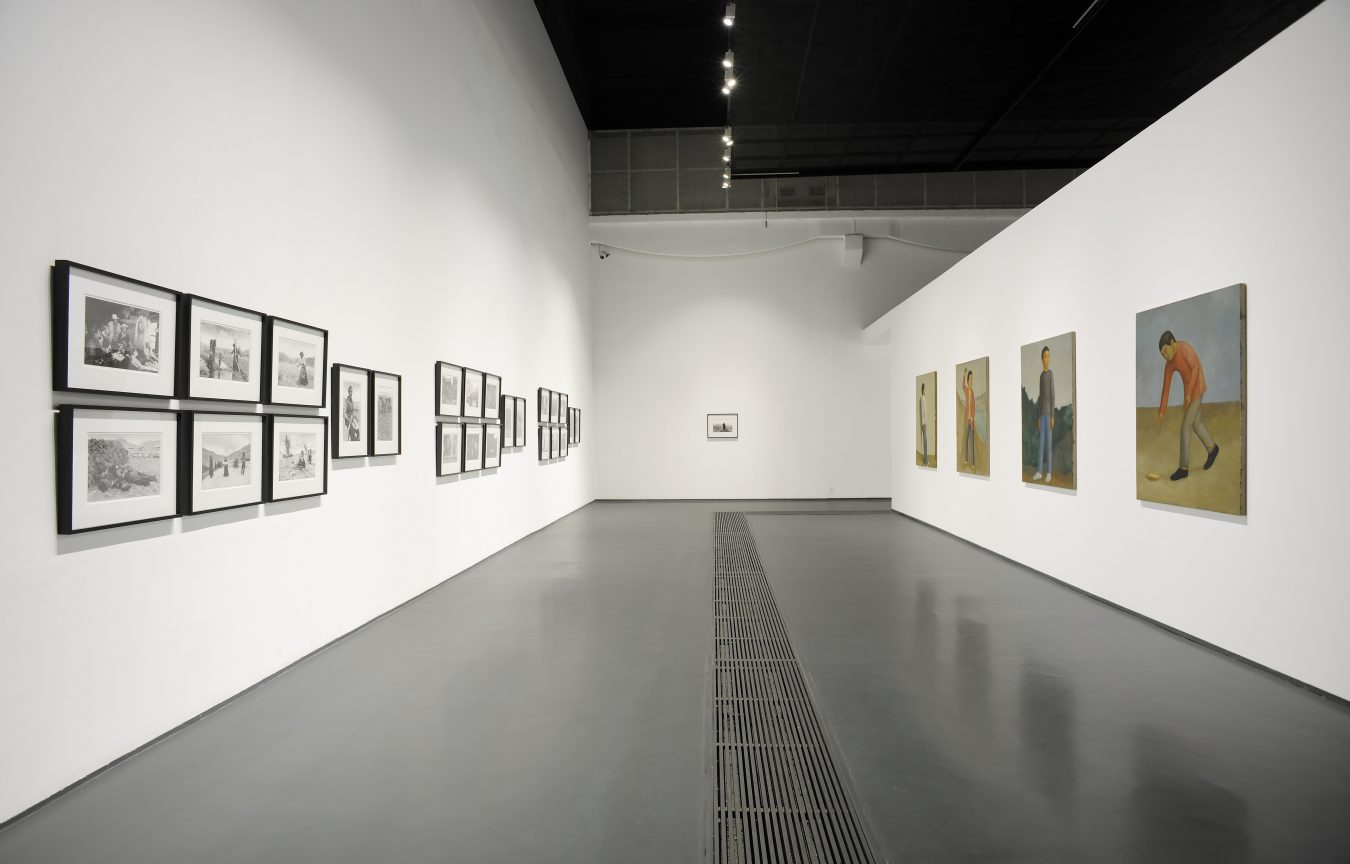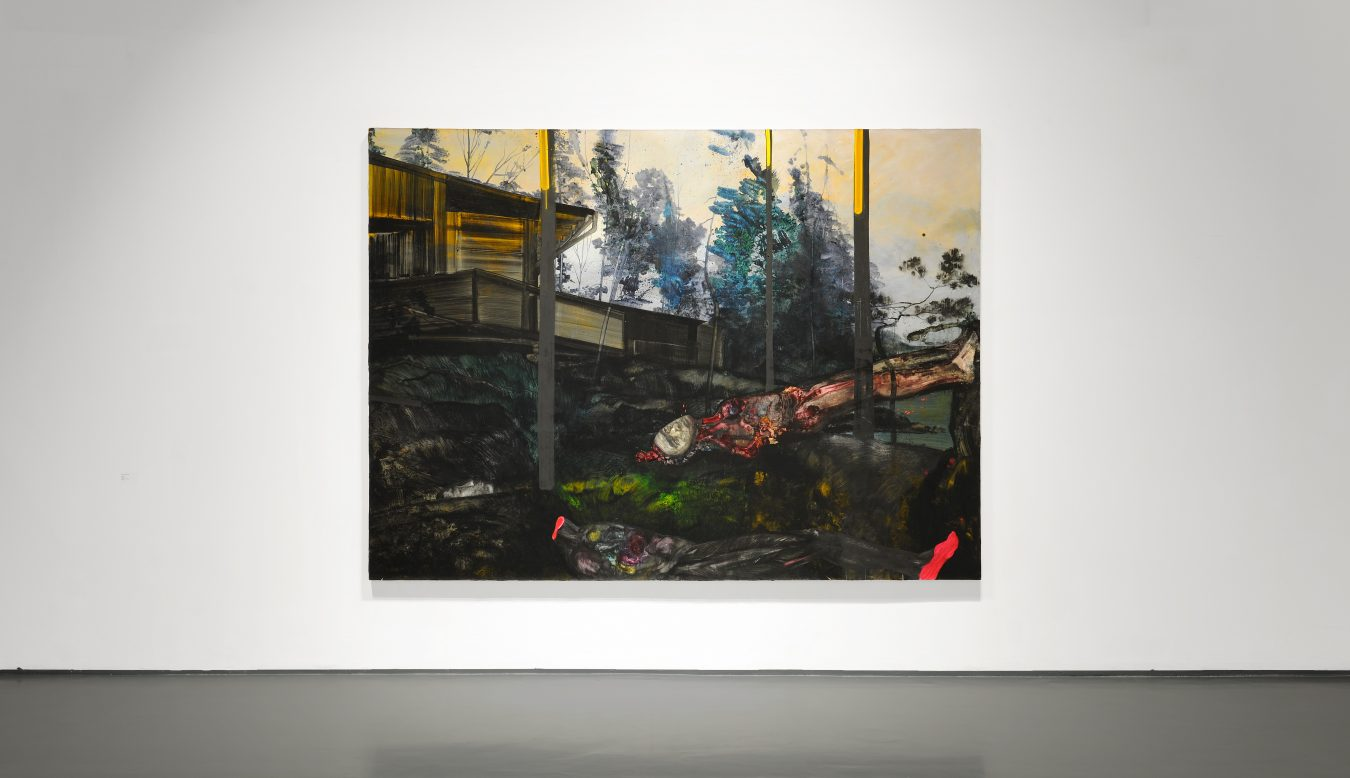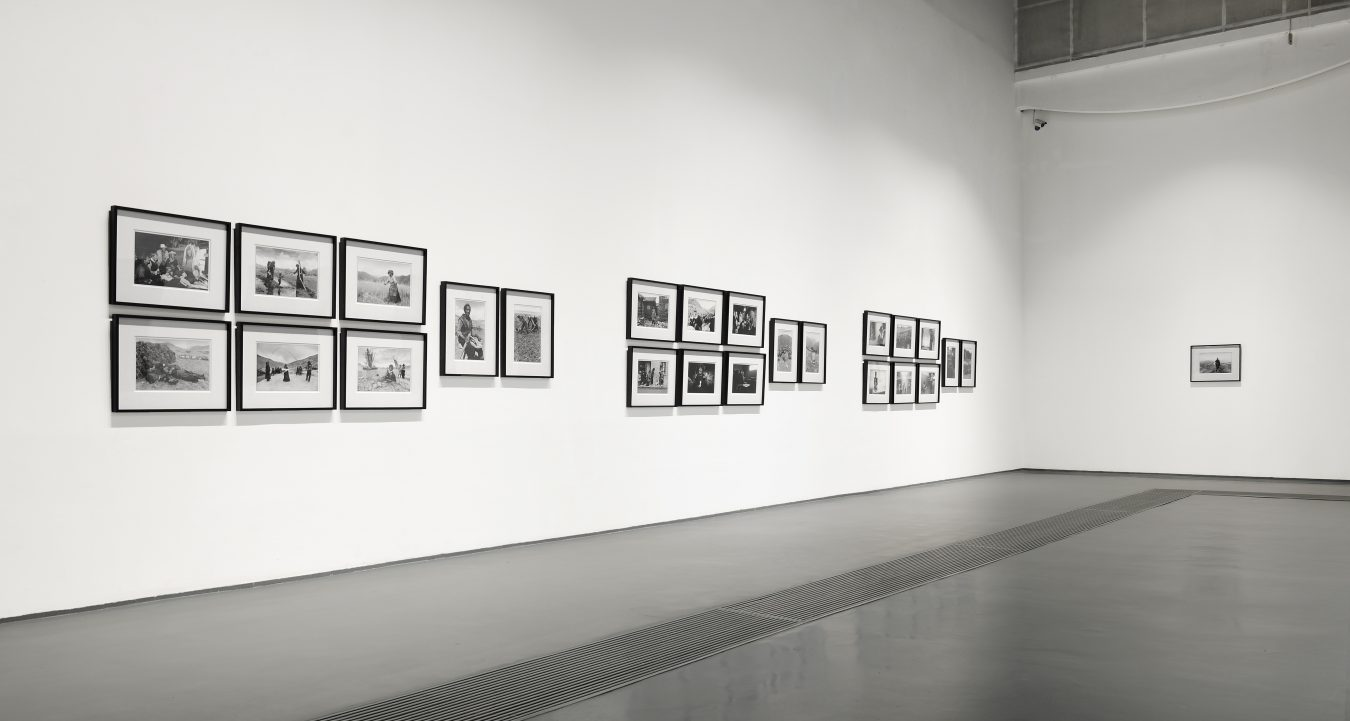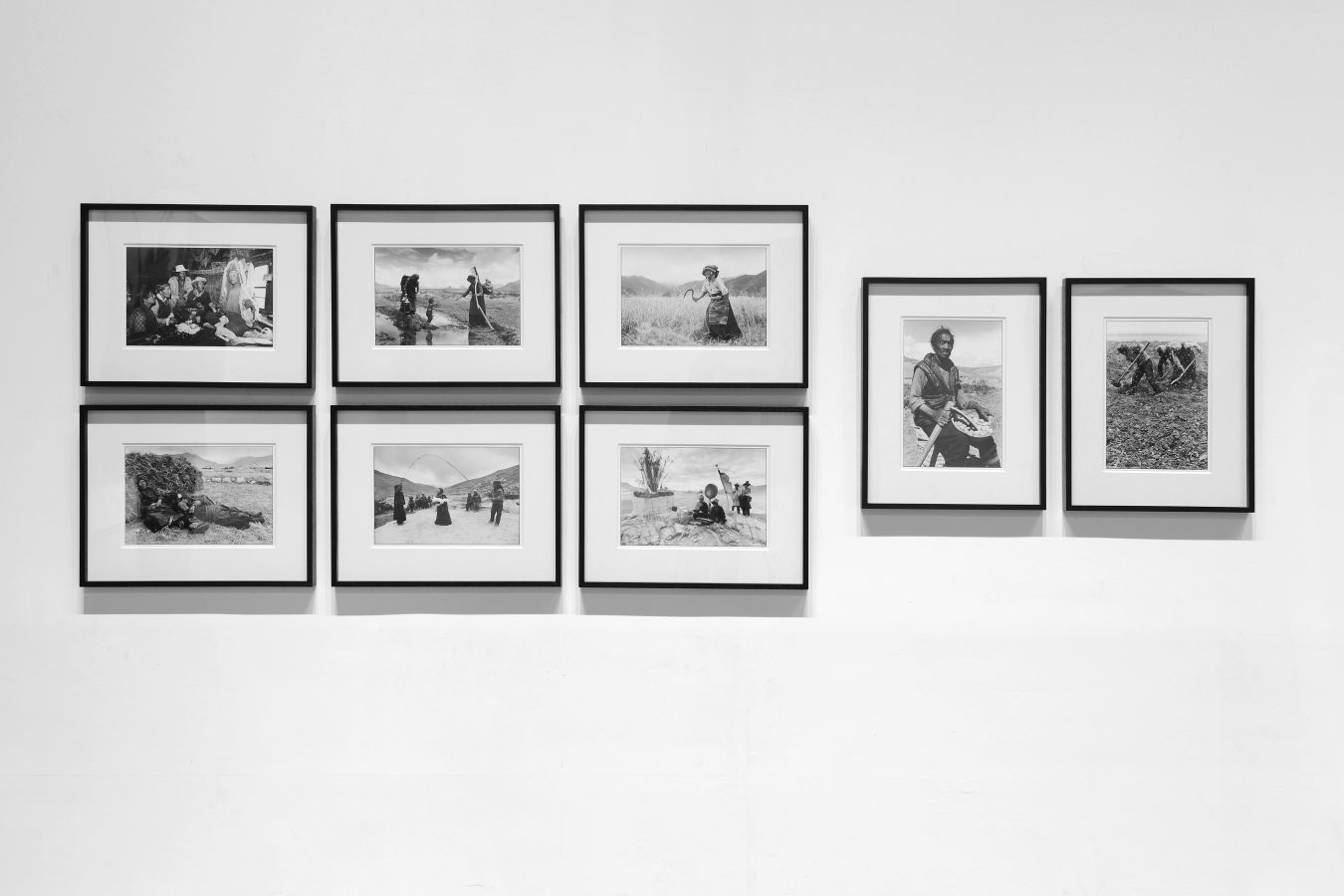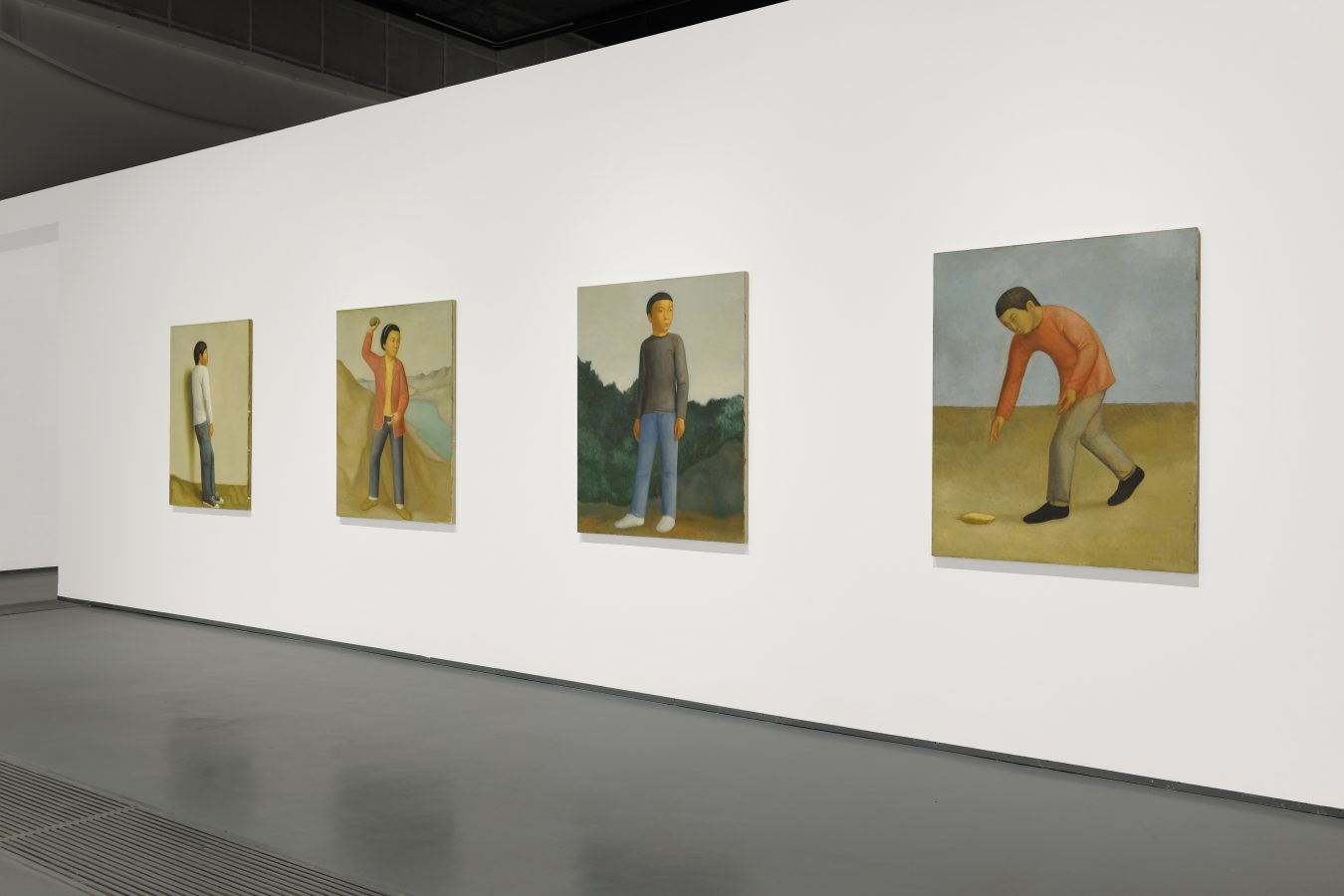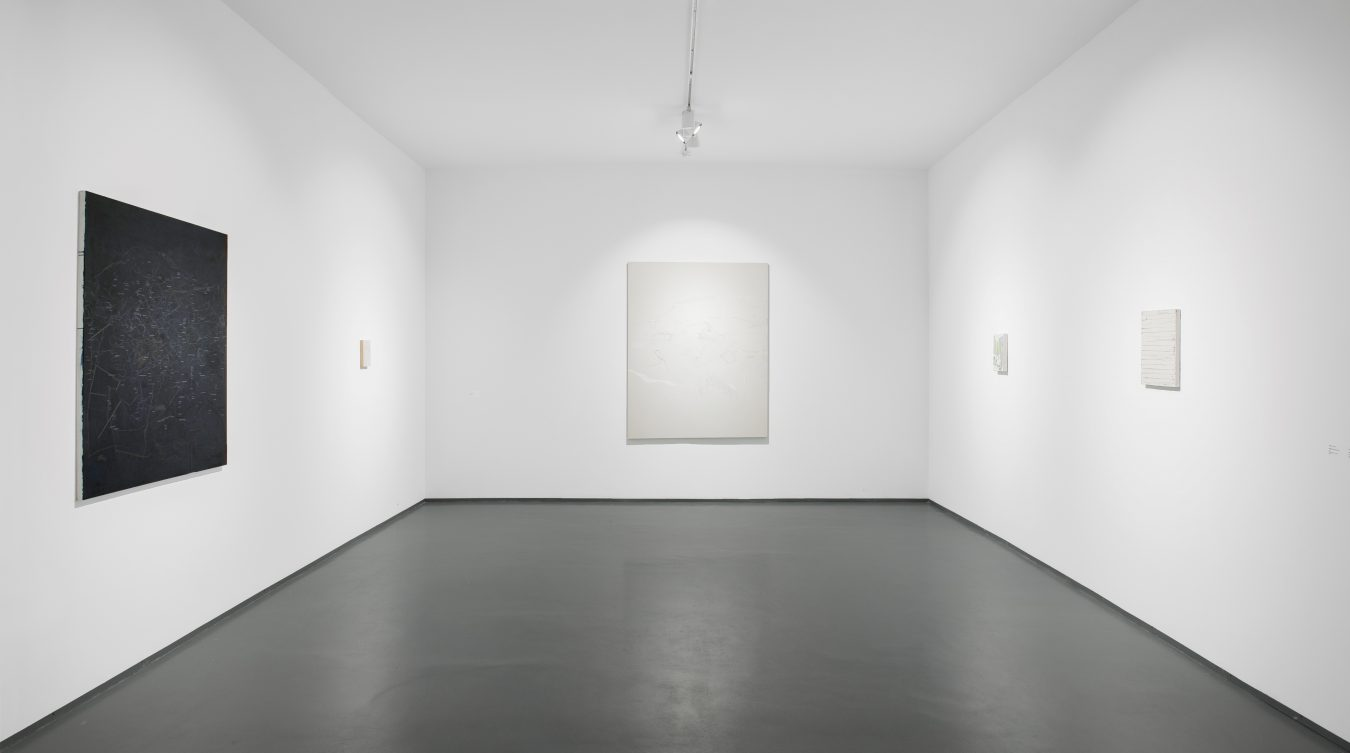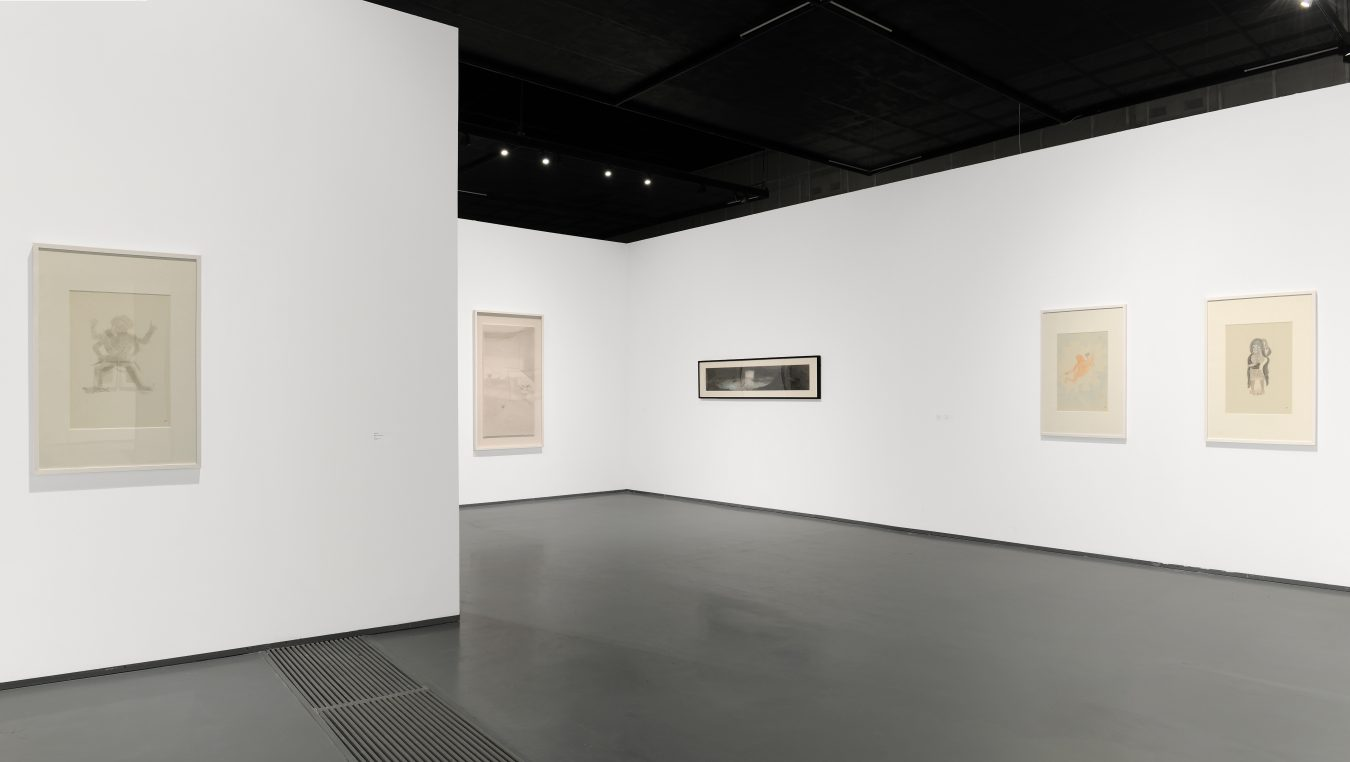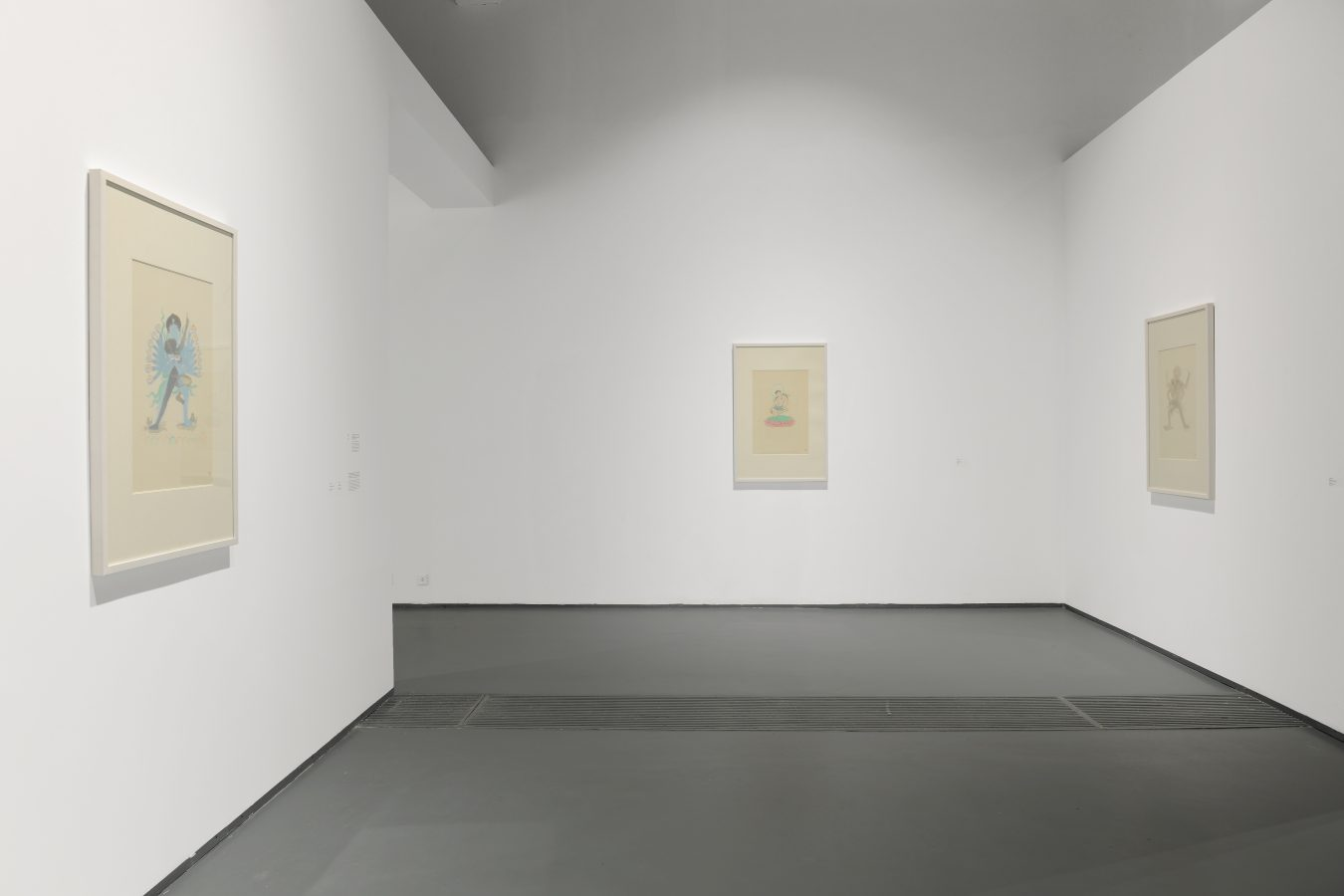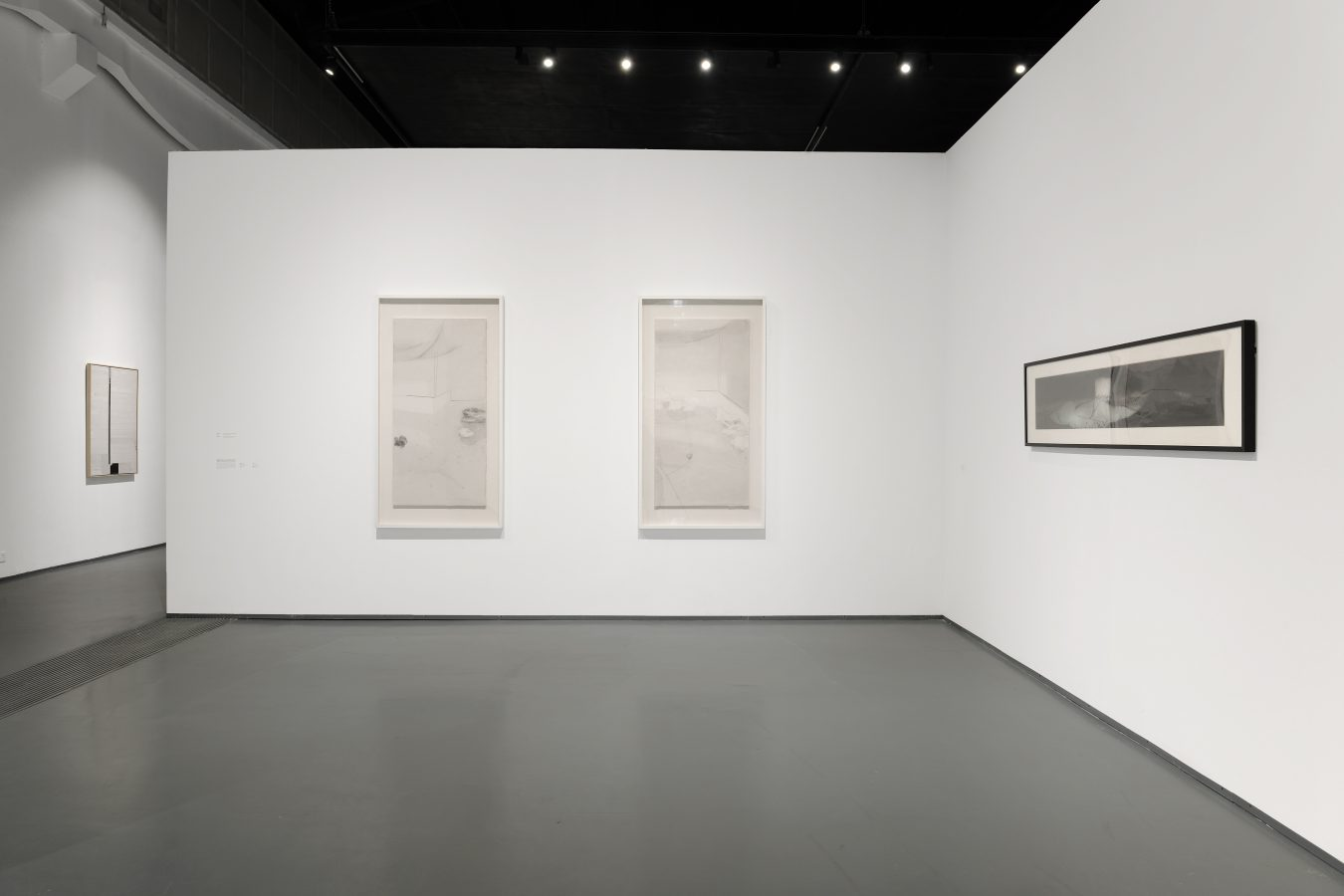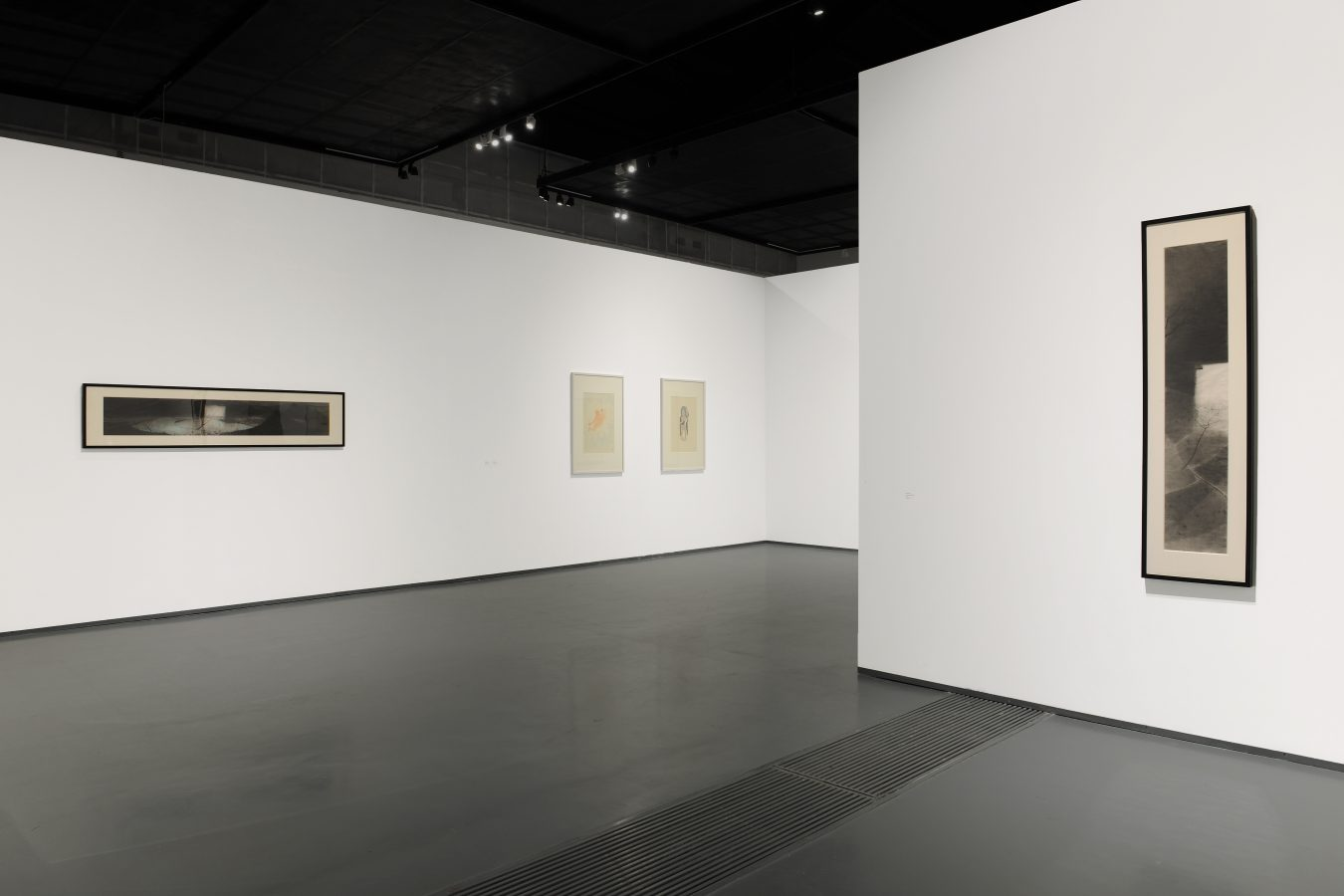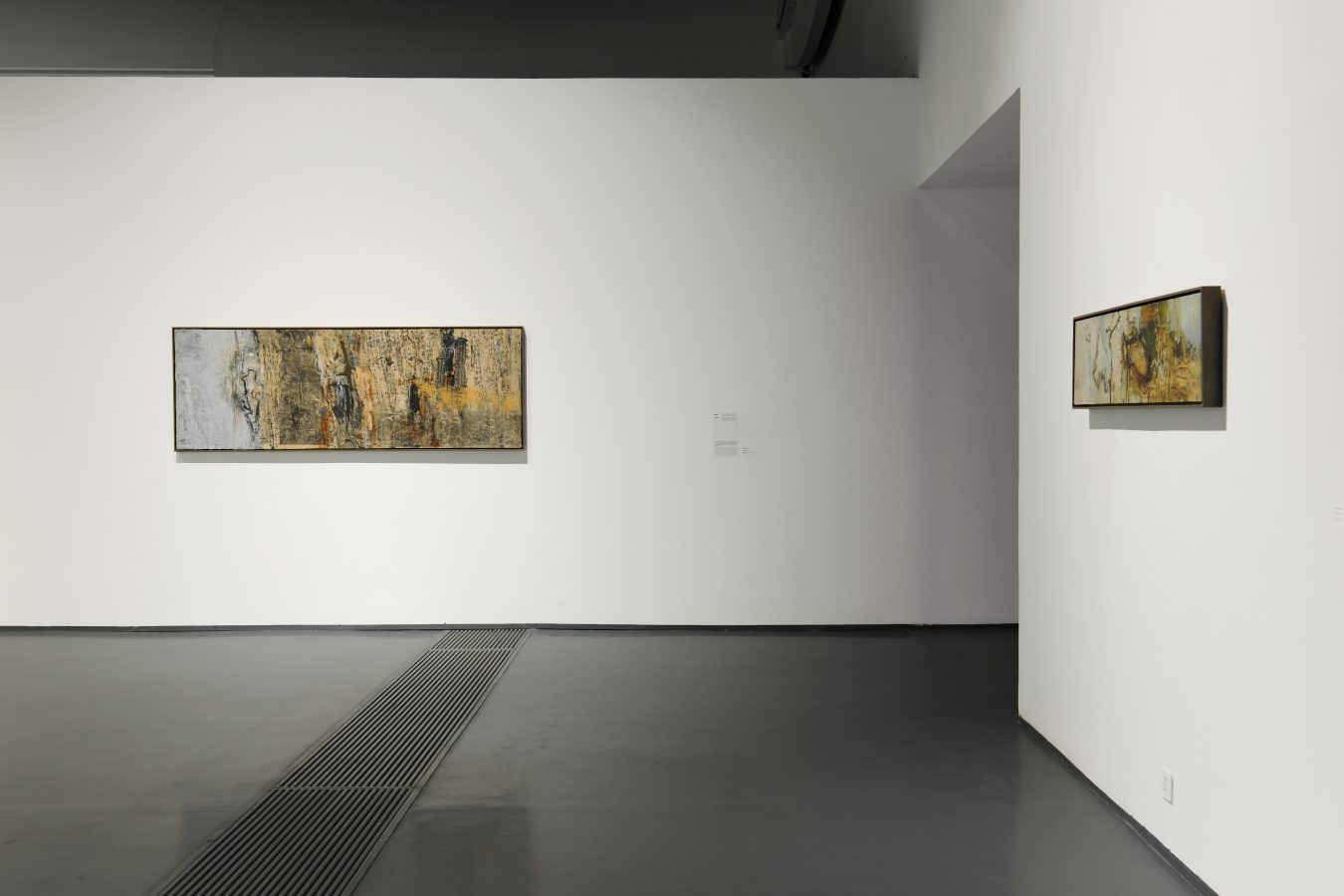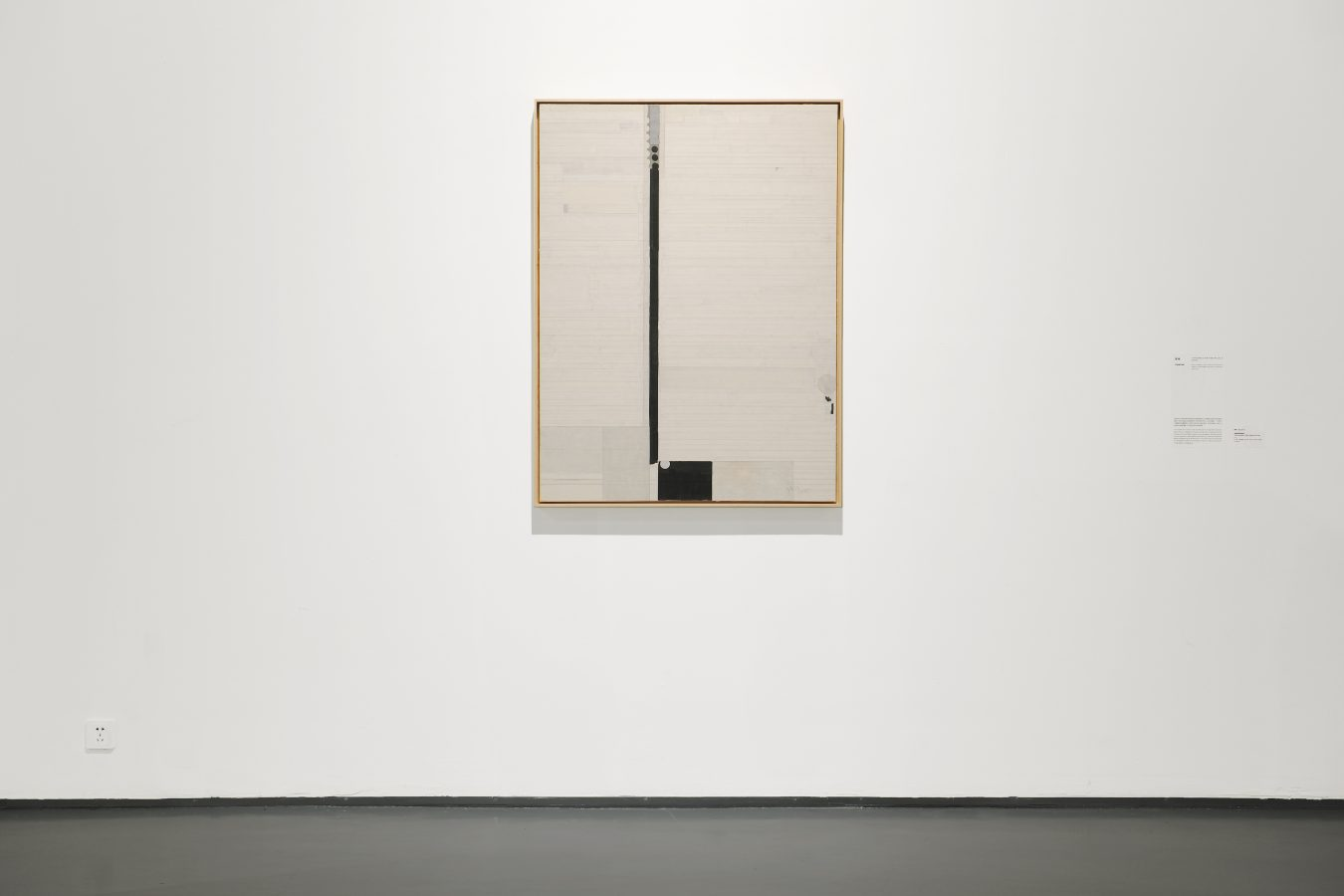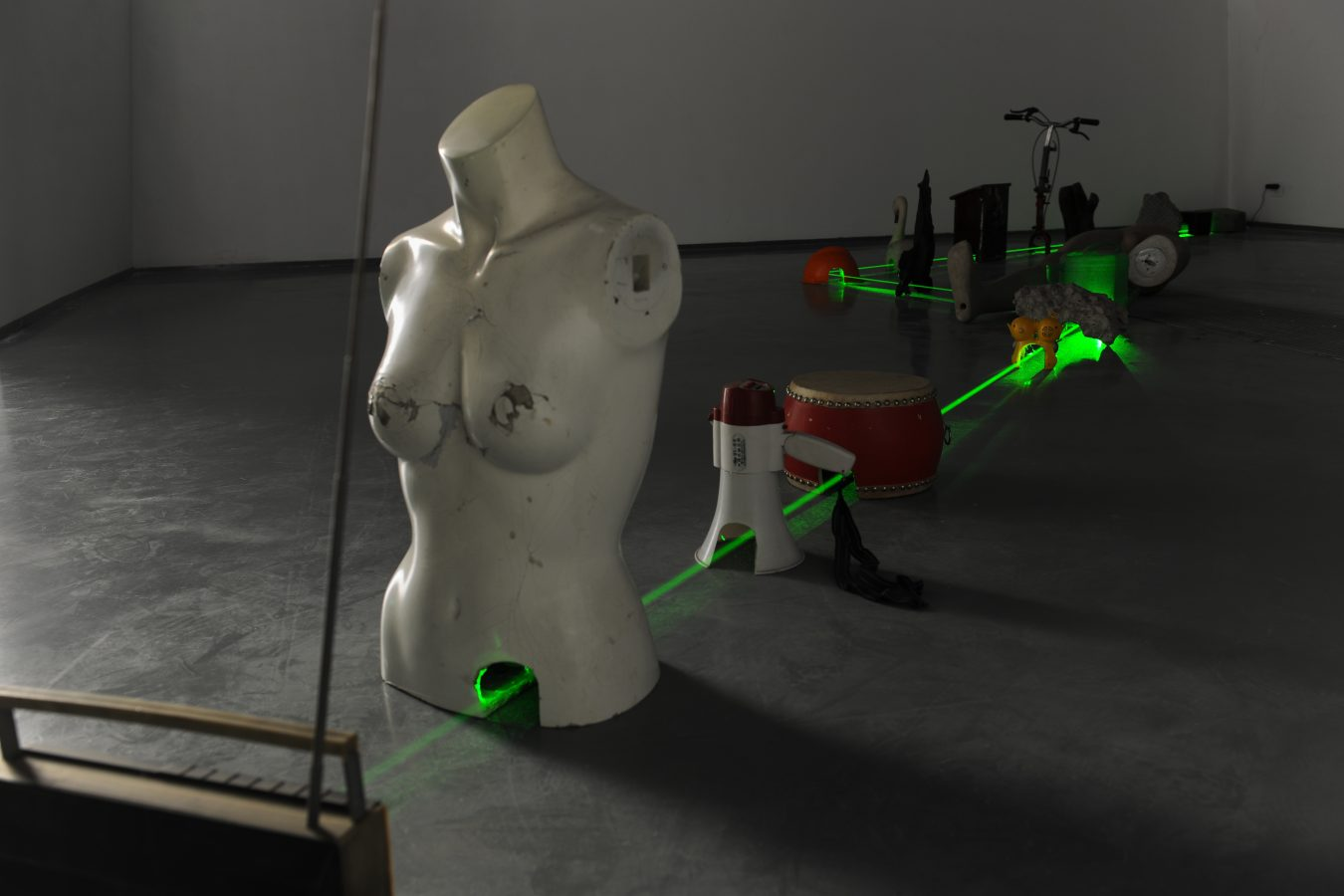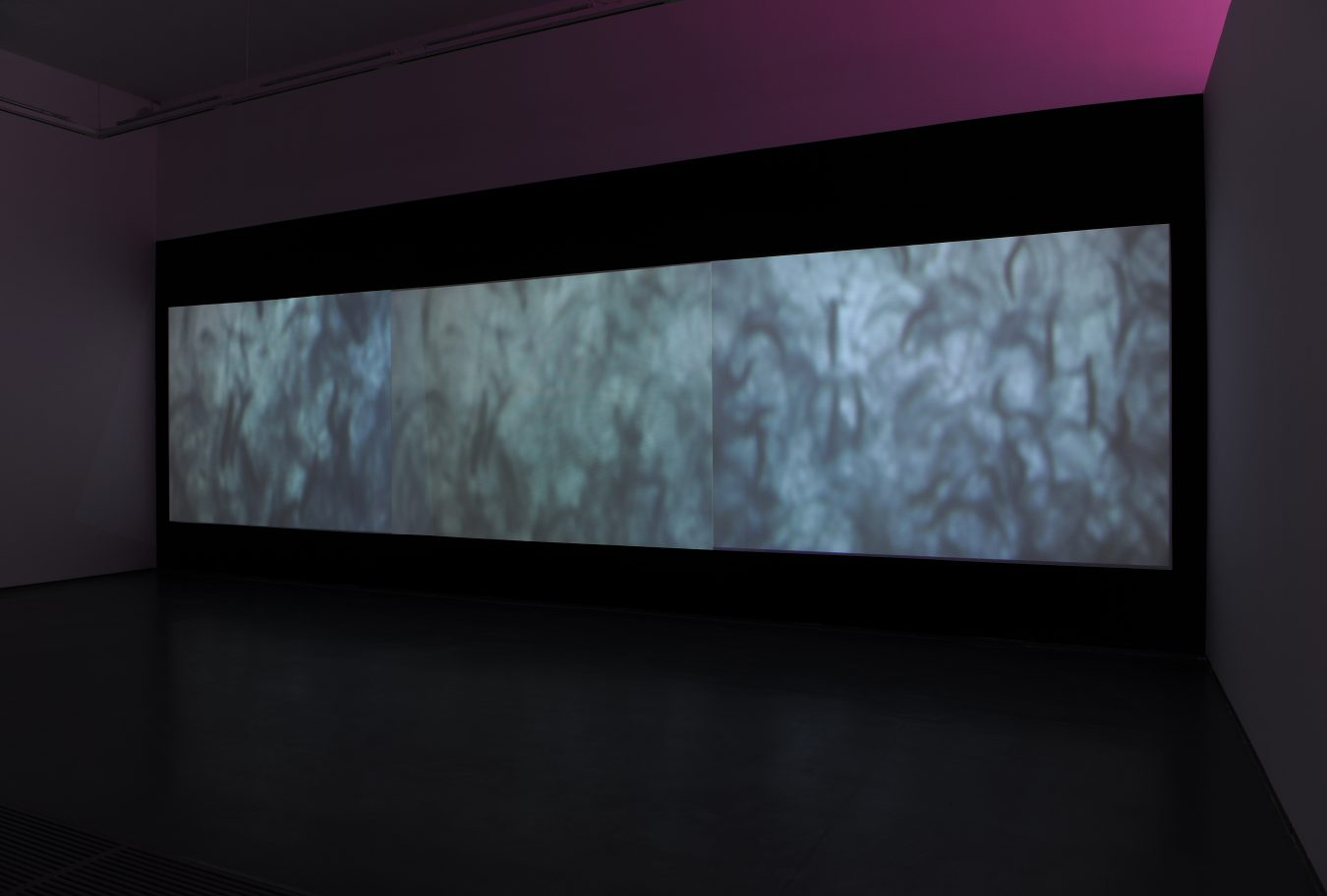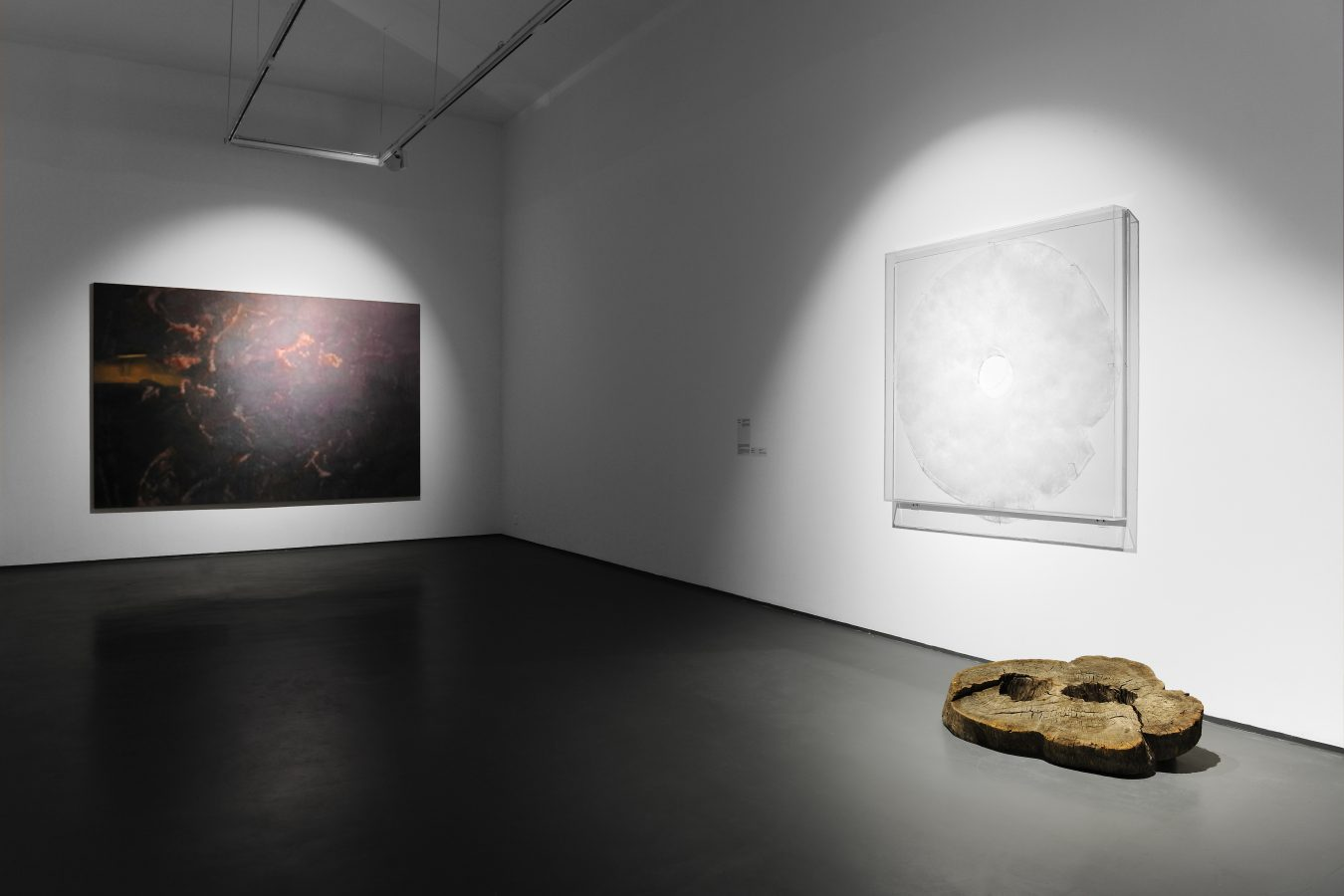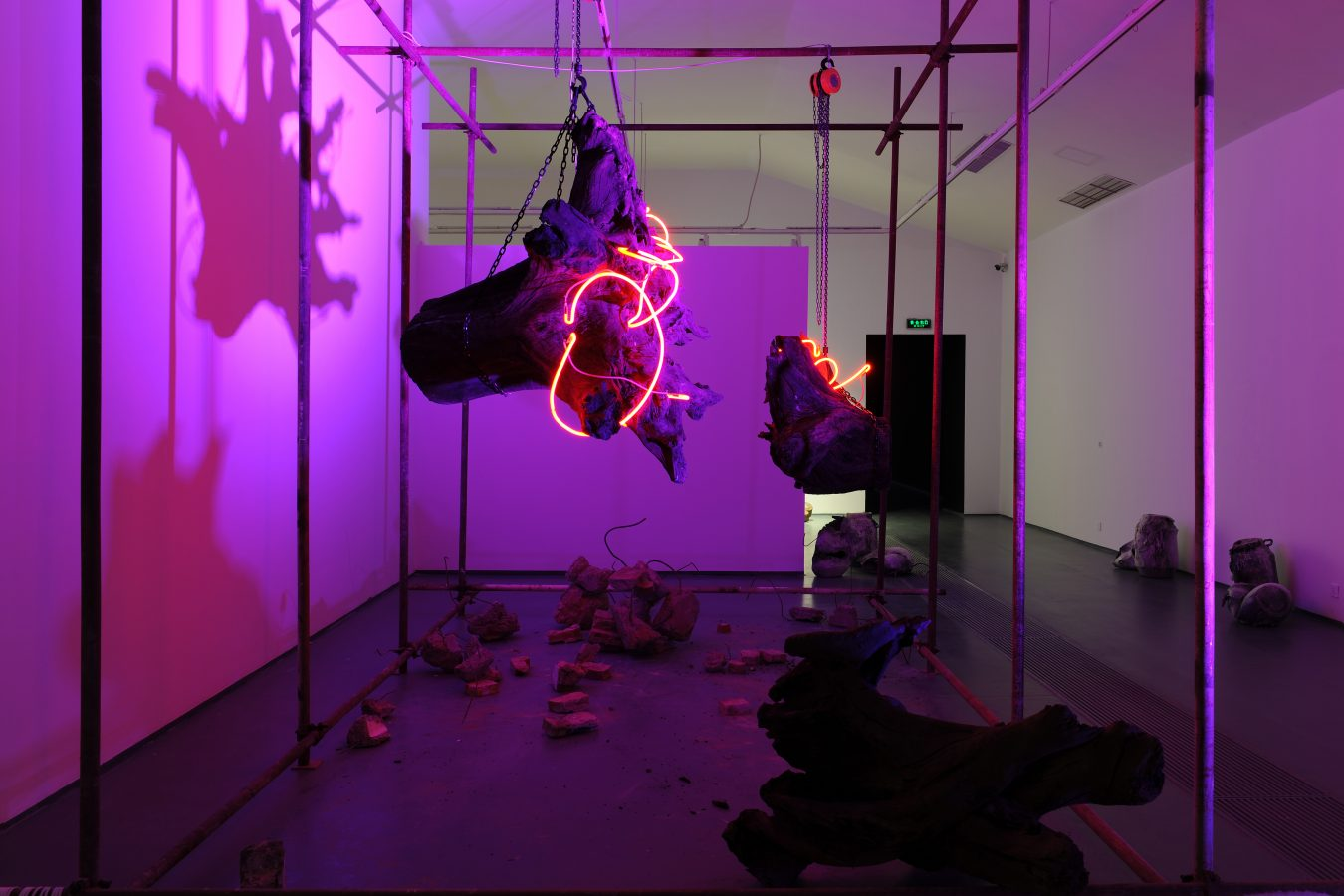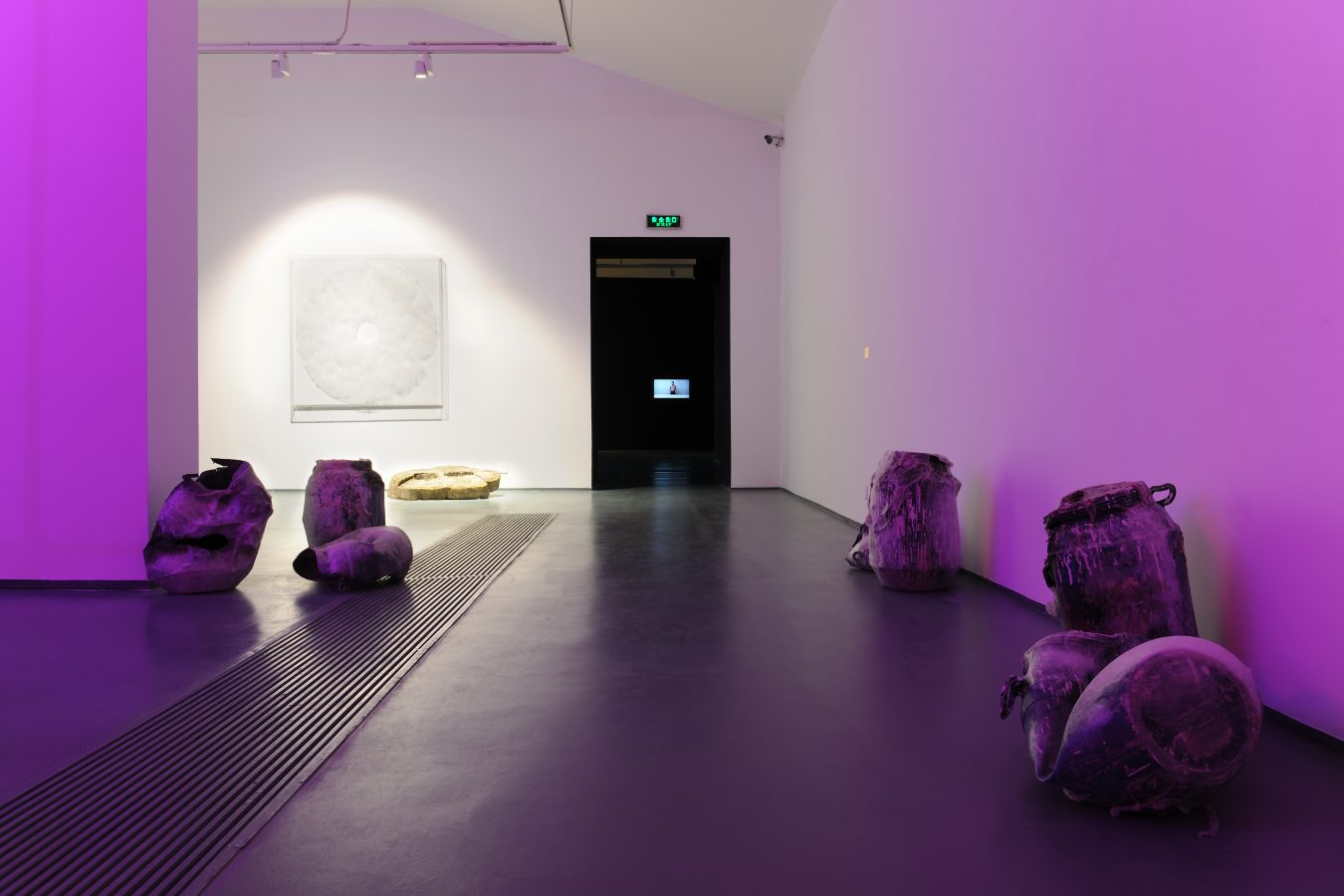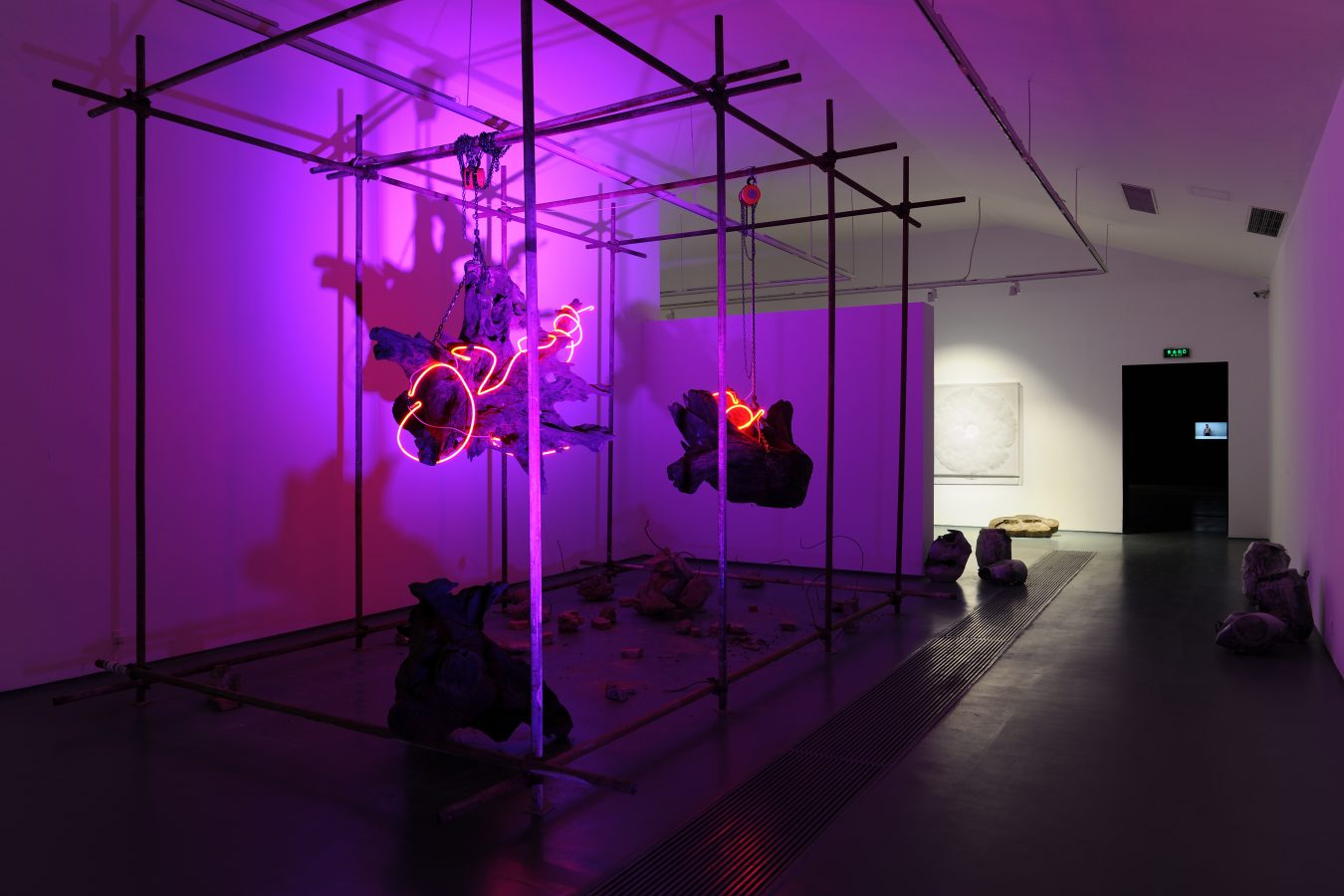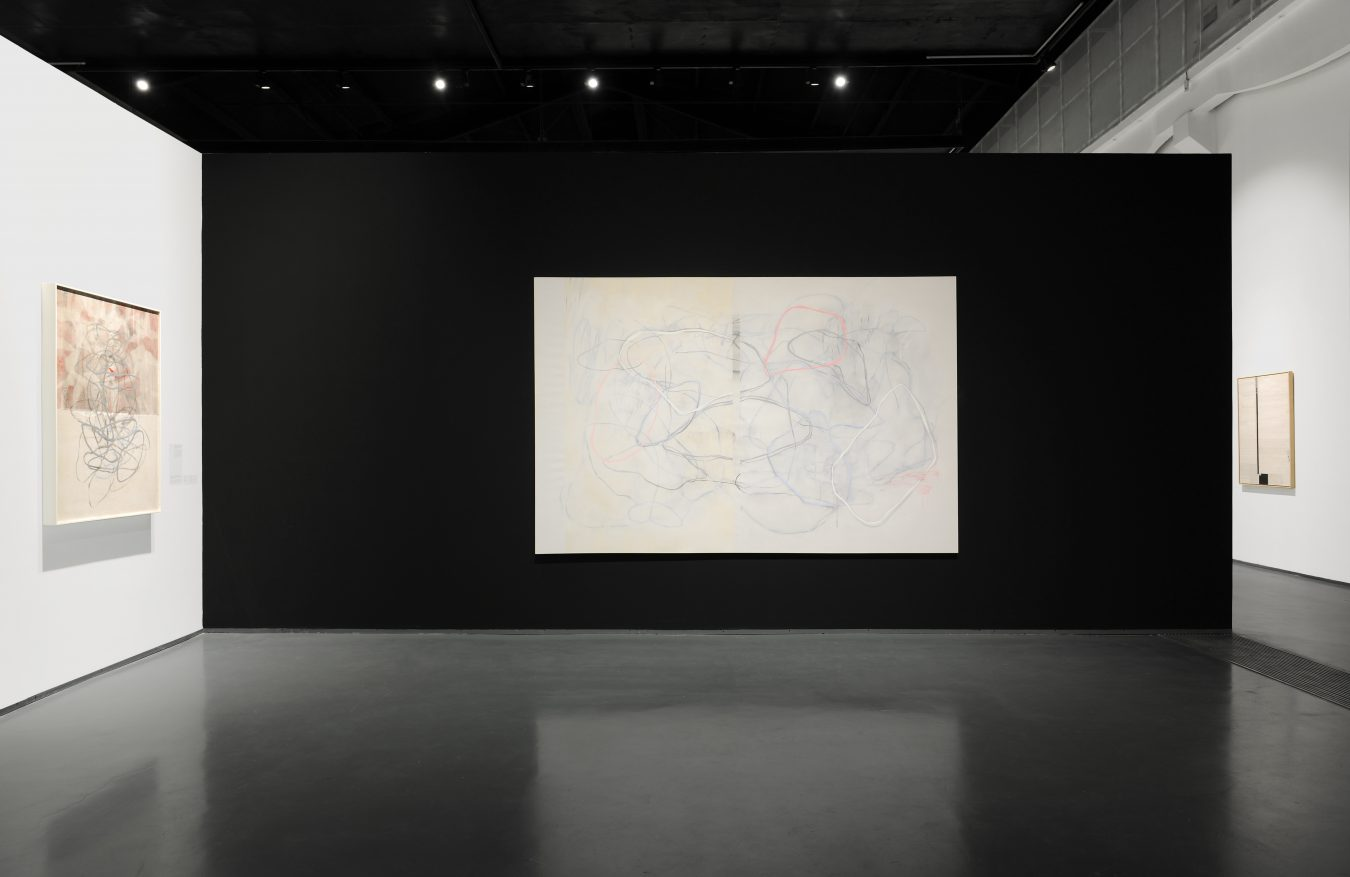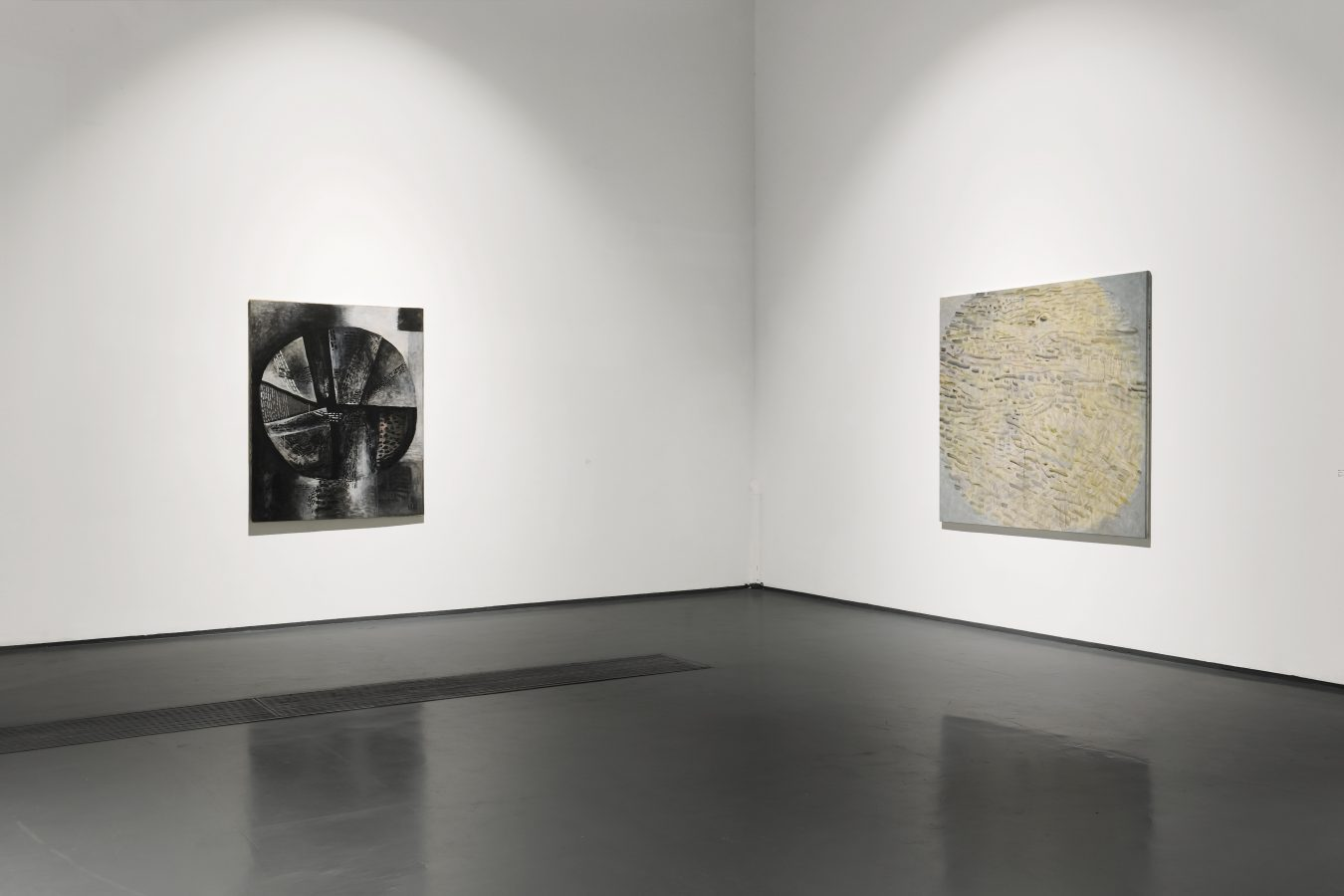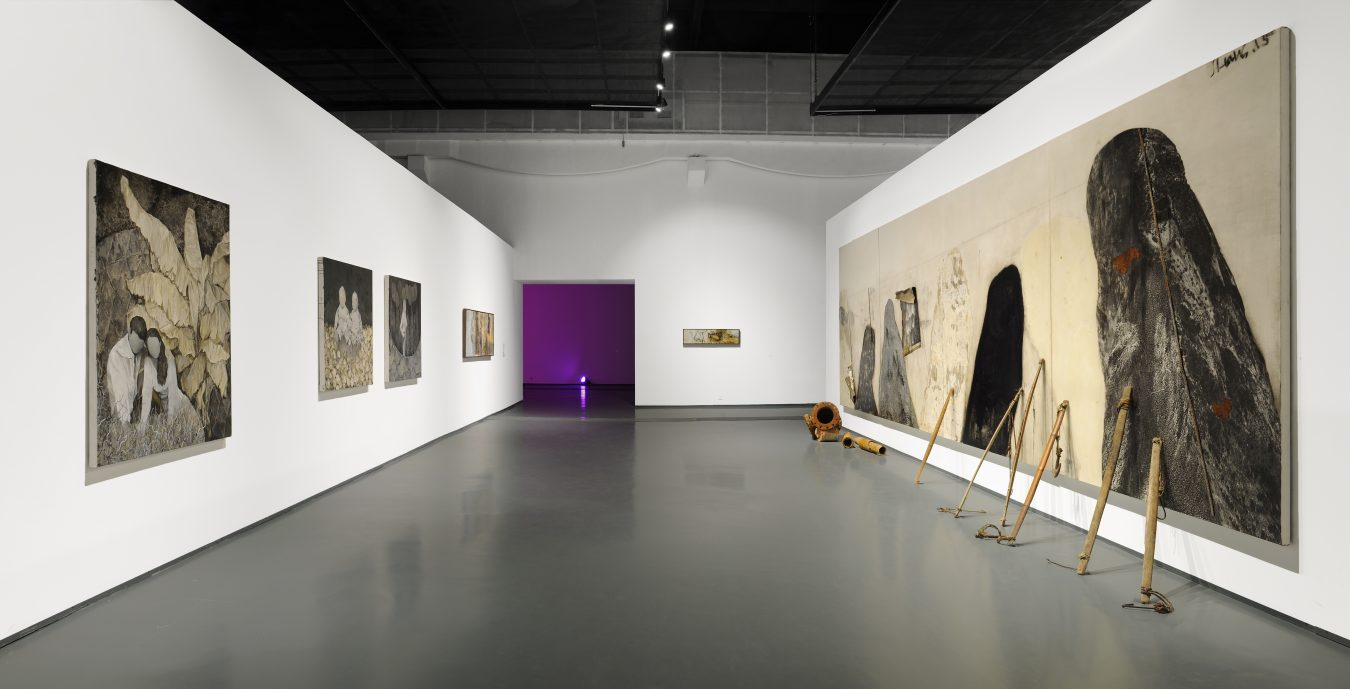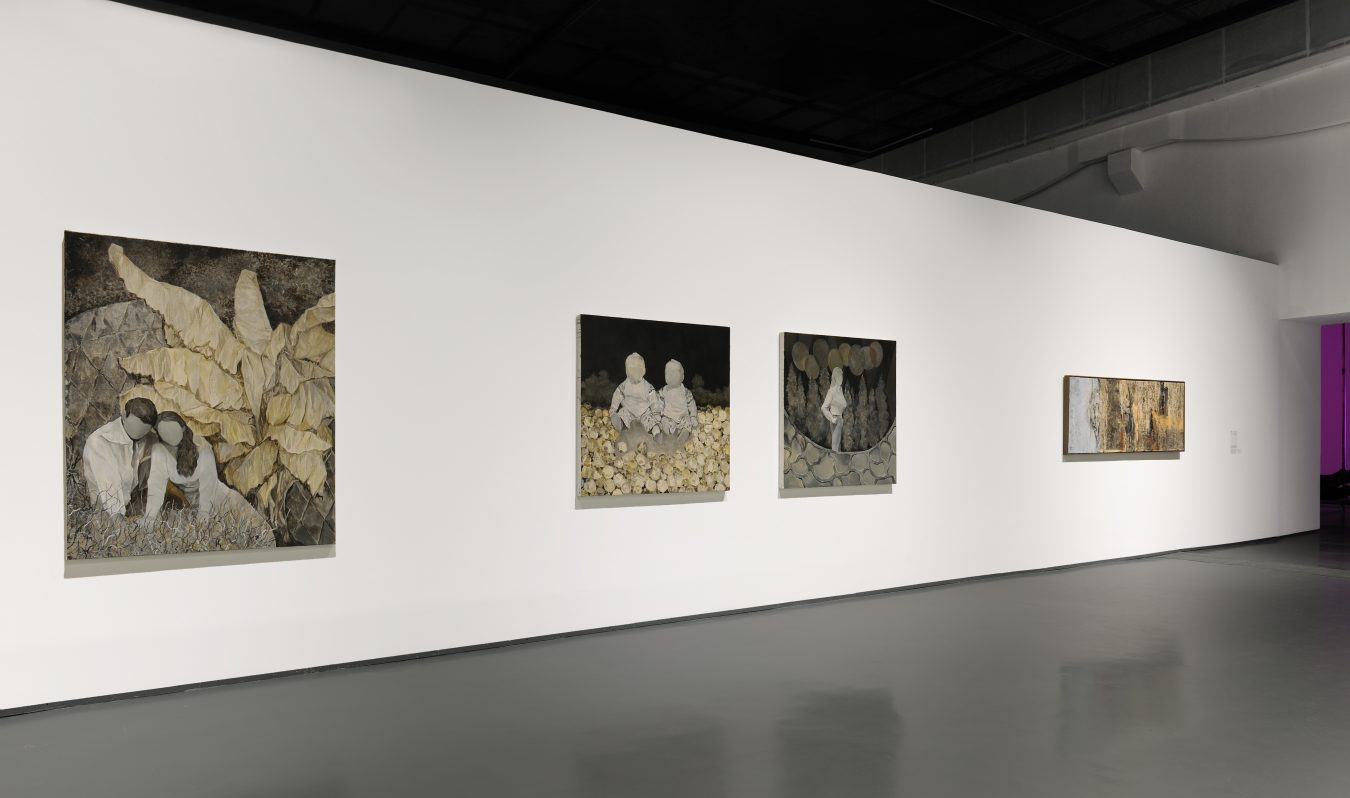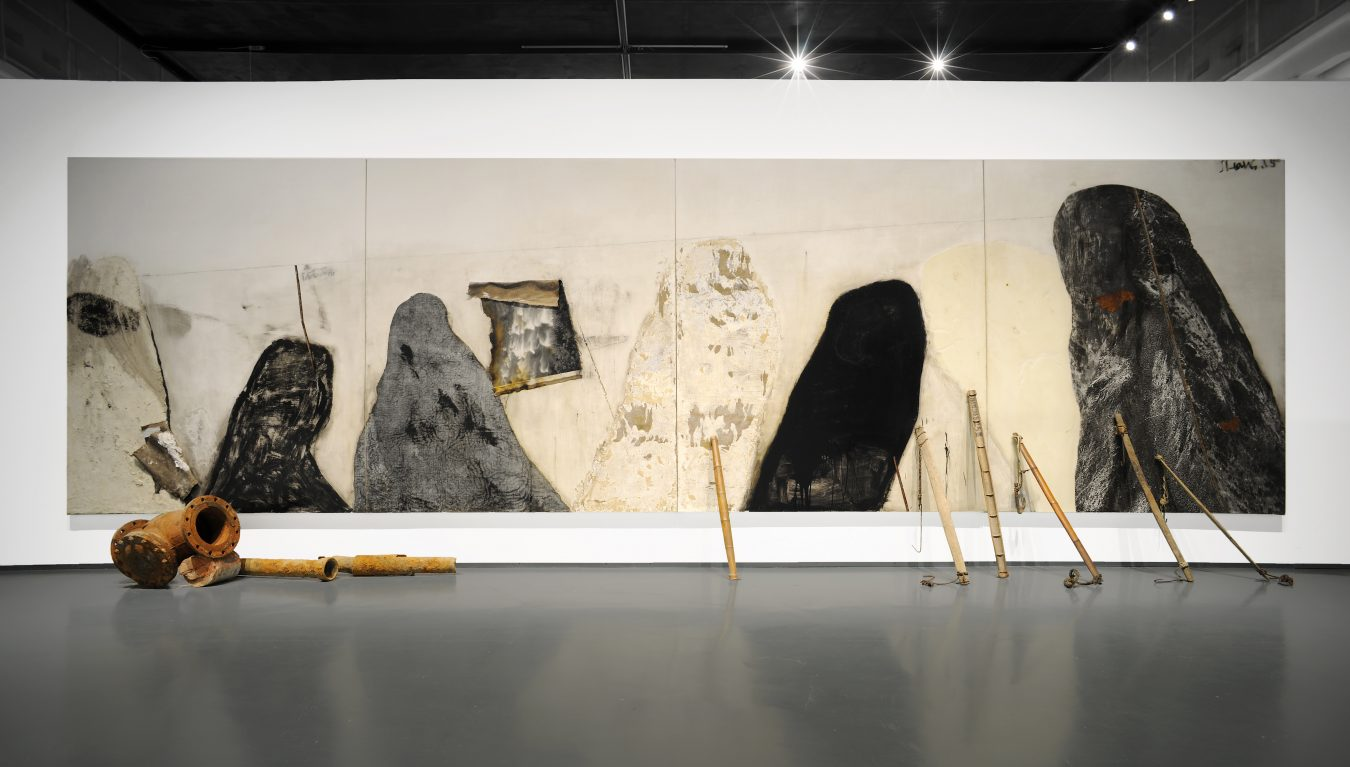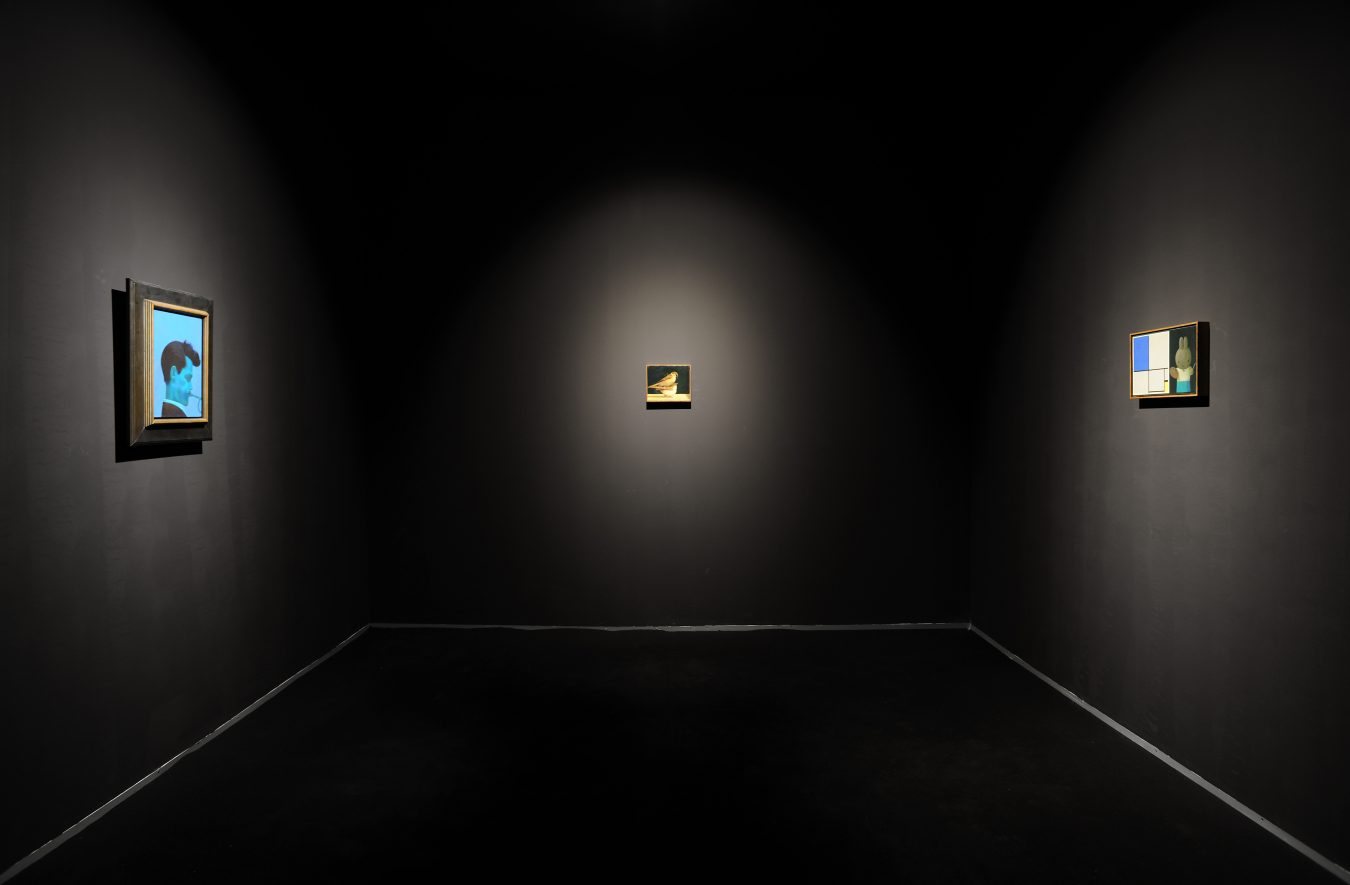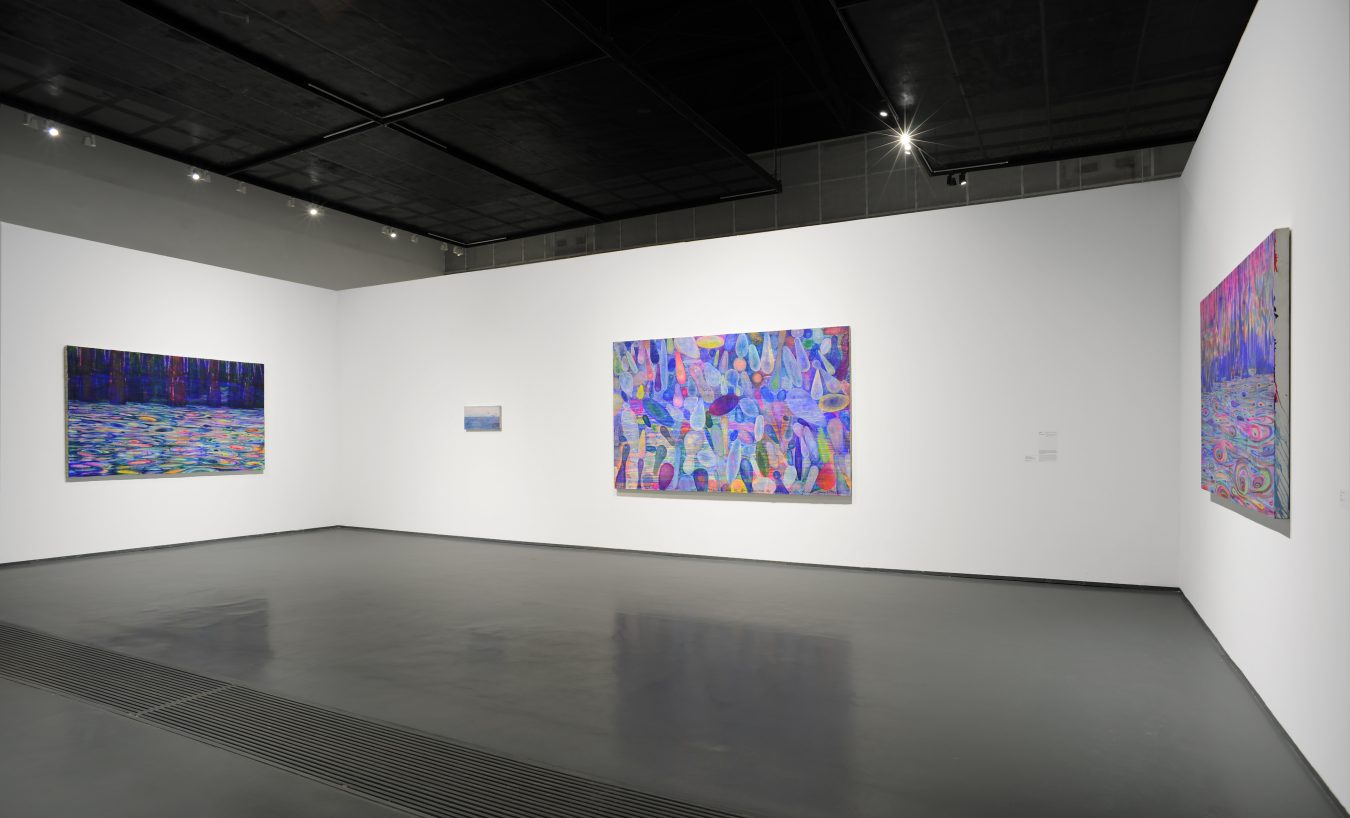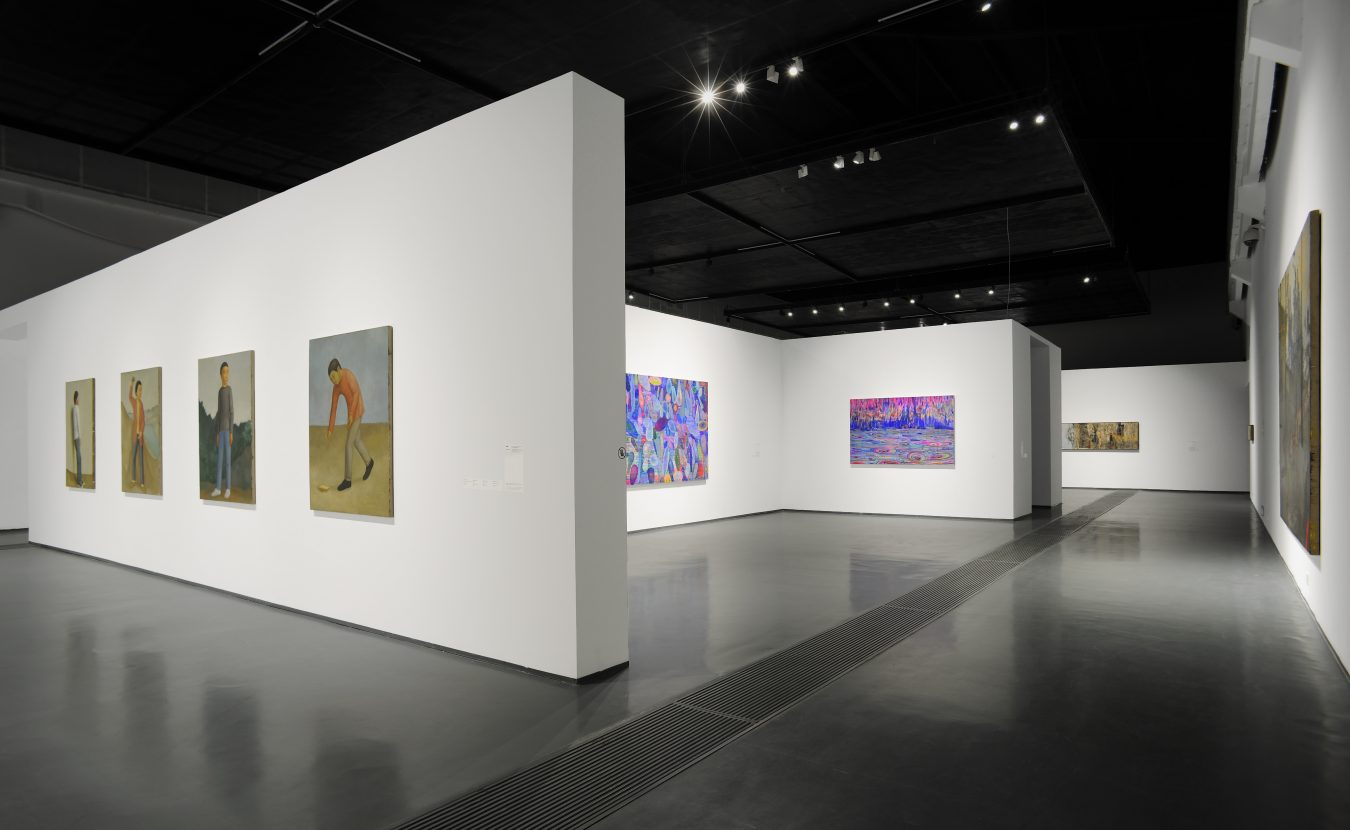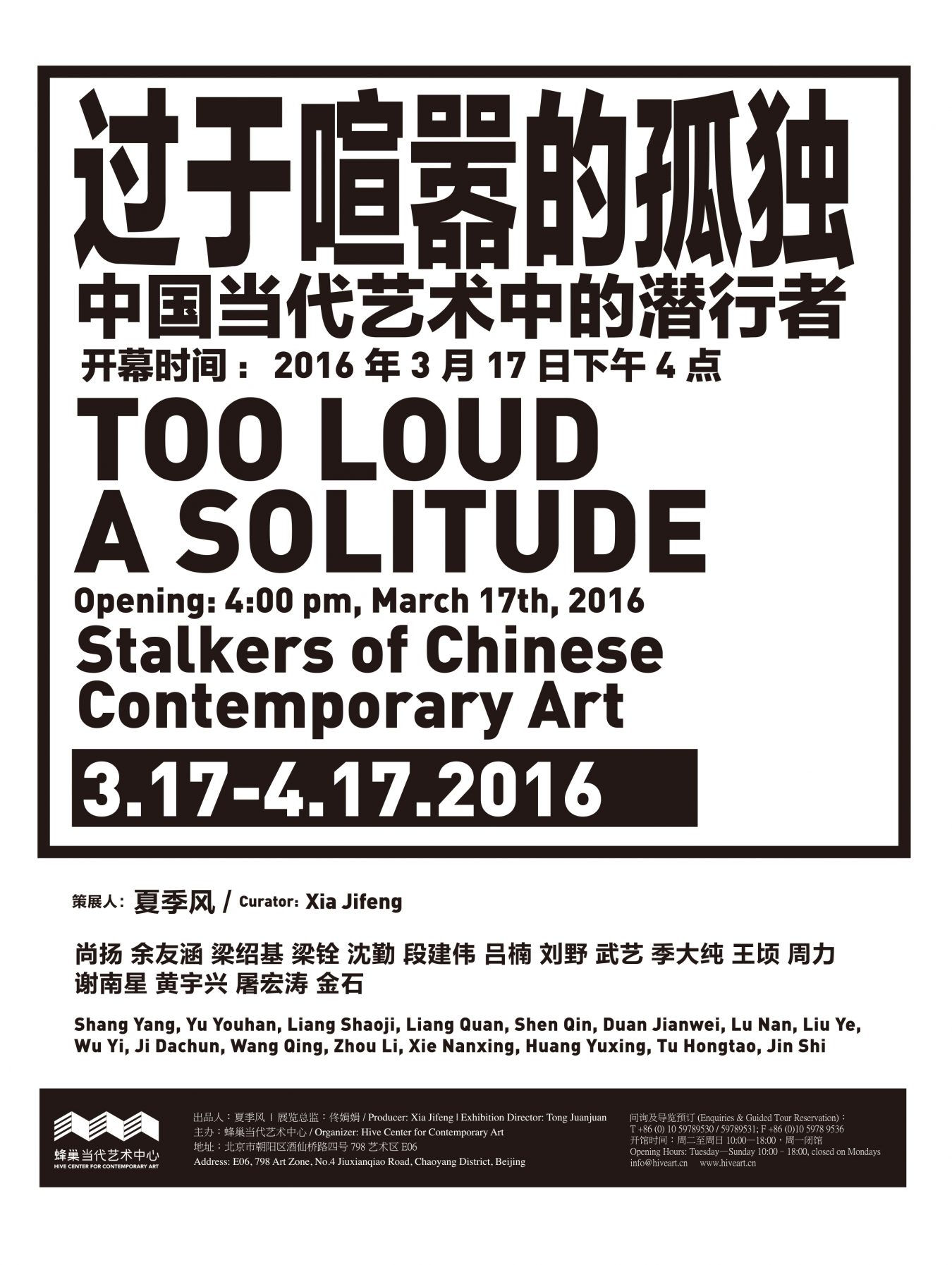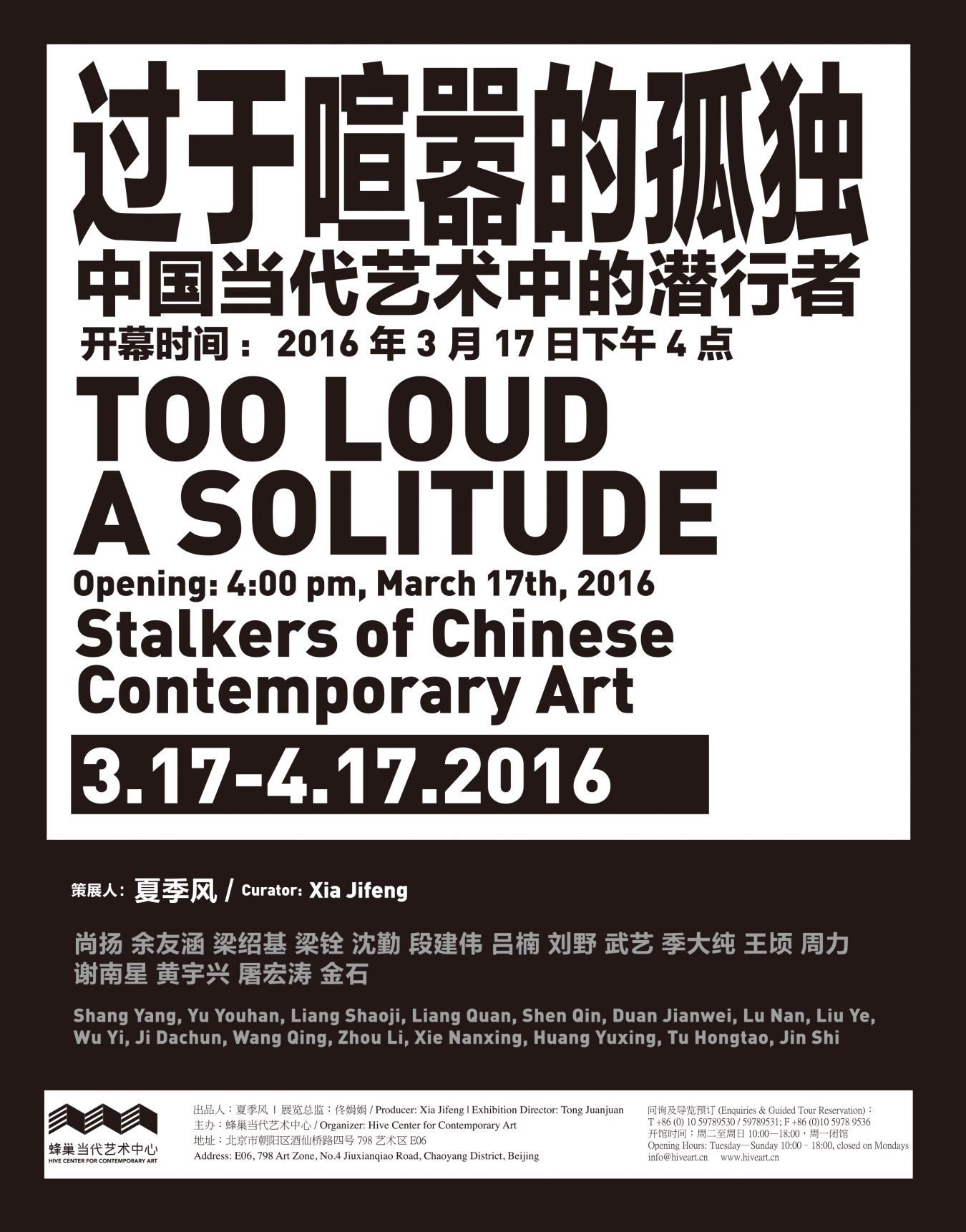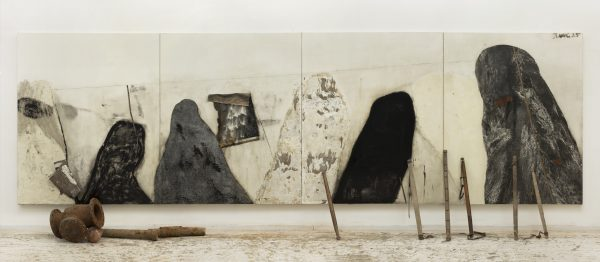2015. 布面综合材料. 现成品及多媒体装置. 253cm×852cm.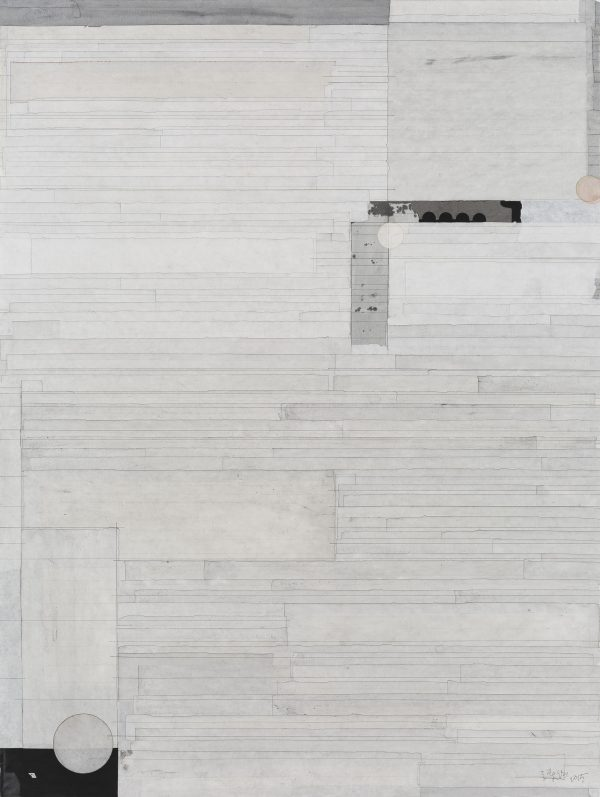Liang Quan

2015. 色、墨、宣纸拼贴. 120×90cm.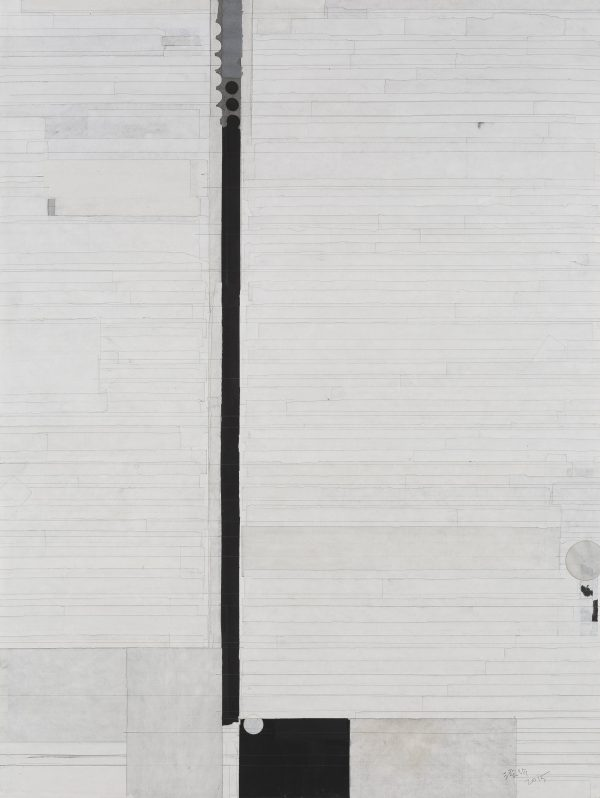2015. 色、墨、宣纸拼贴. 120×90cm.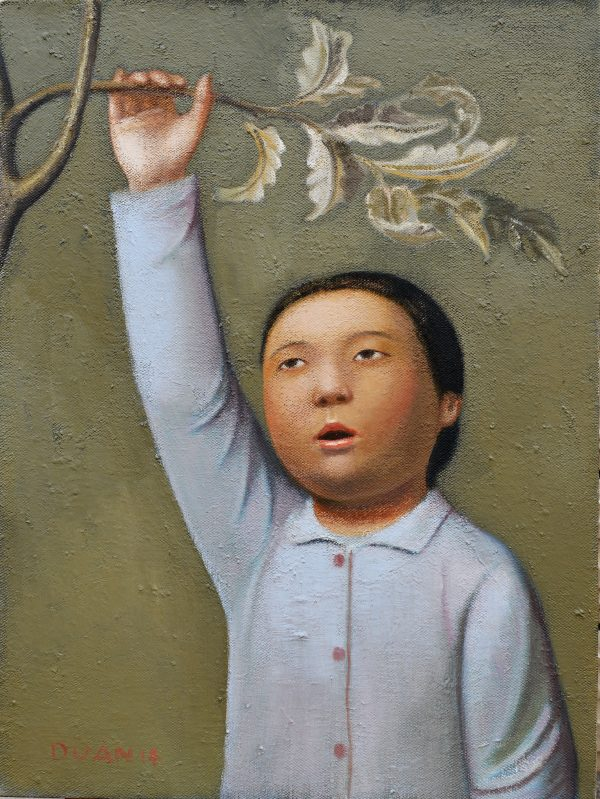2014. 布面油画. 60×45cm.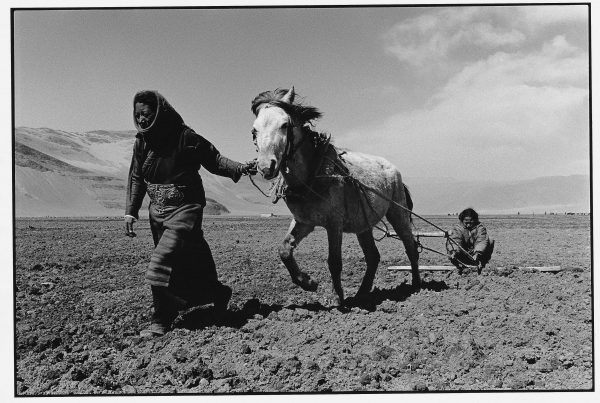2004. 银盐纸基. 27.1×40.6cm. 40.6×50.8cm framed.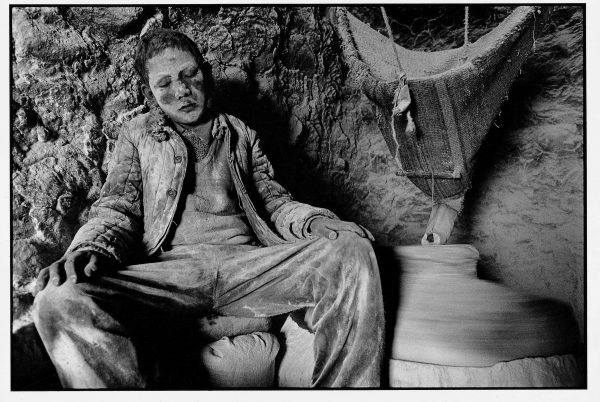2003. 银盐纸基. 27.1×40.6cm. 40.6×50.8cm framed.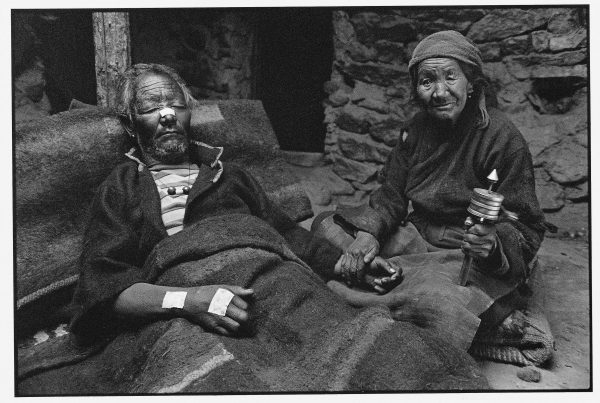2001. 银盐纸基. 27.1×40.6cm. 40.6×50.8cm framed.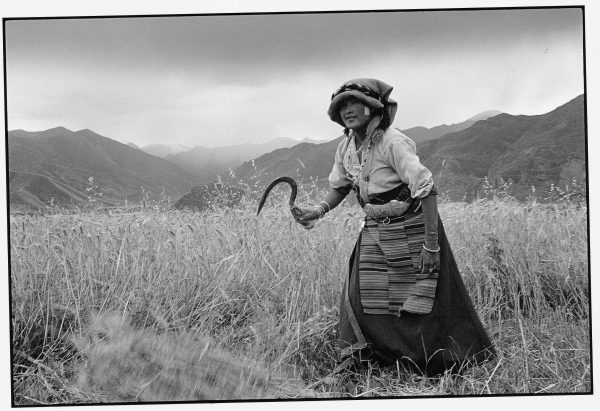2000. 银盐纸基. 27.1×40.6cm. 40.6×50.8cm framed.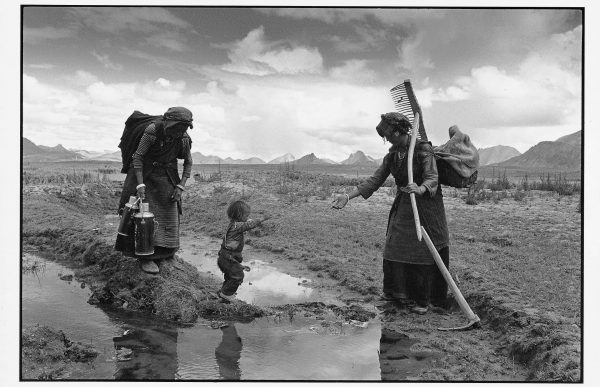1999. 银盐纸基. 27.1×40.6cm. 40.6×50.8cm framed.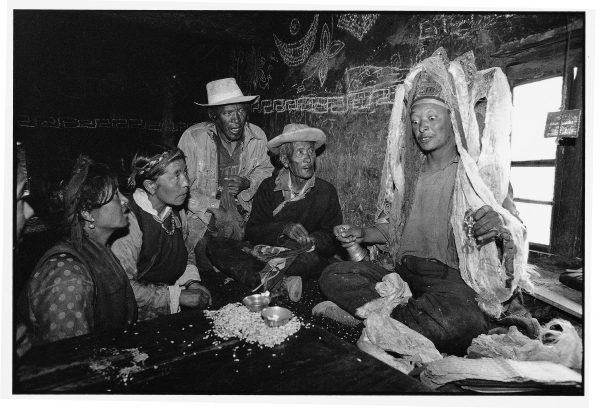1997. 银盐纸基. 27.1×40.6cm. 40.6×50.8cm framed.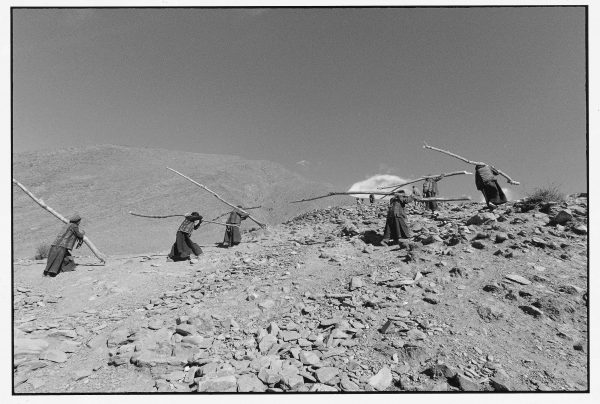1997. 银盐纸基. 27.1×40.6cm. 40.6×50.8cm framed.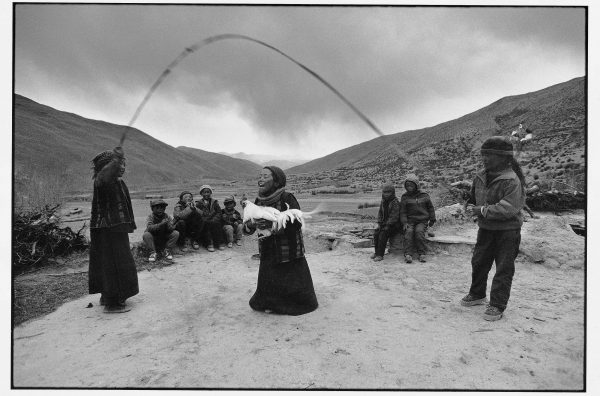1996. 银盐纸基. 27.1×40.6cm. 40.6×50.8cm framed.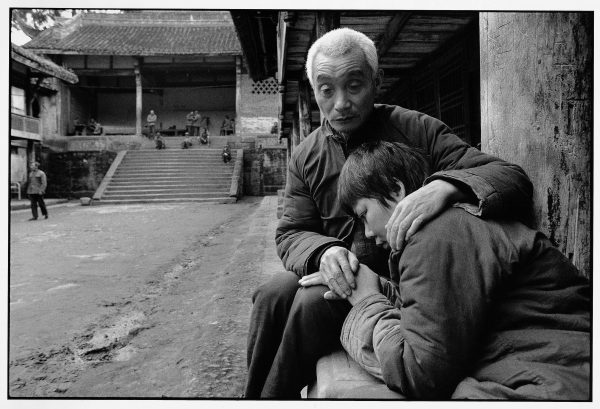1990. 银盐纸基. 27.1×40.6cm. 40.6×50.8cm framed.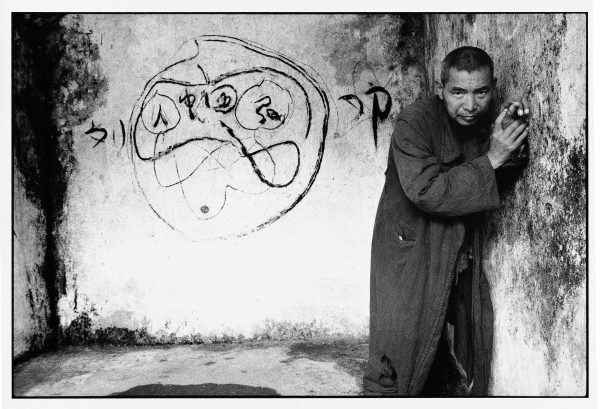1990. 银盐纸基. 27.1×40.6cm. 40.6×50.8cm framed.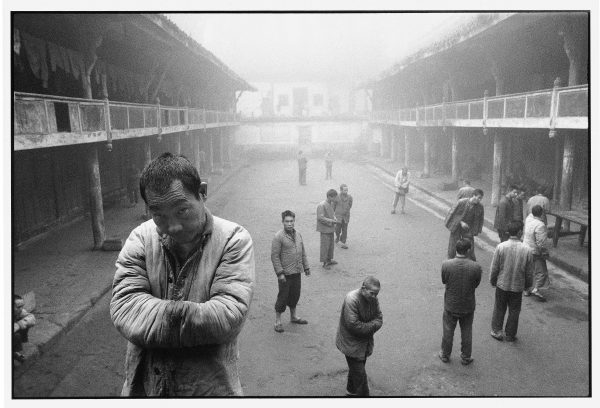1990. 银盐纸基. 27.1×40.6cm. 40.6×50.8cm framed.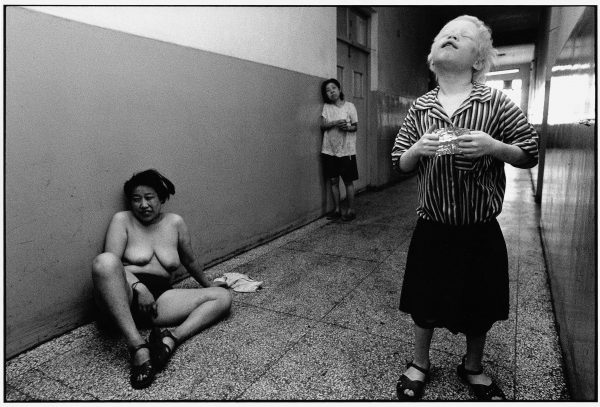1989. 银盐纸基. 27.1×40.6cm. 40.6×50.8cm framed.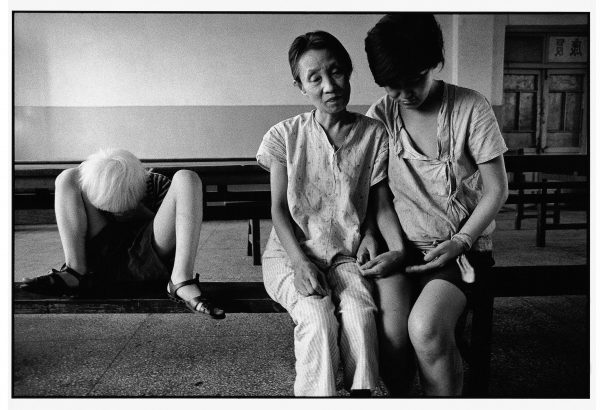1989. 银盐纸基. 27.1×40.6cm. 40.6×50.8cm framed.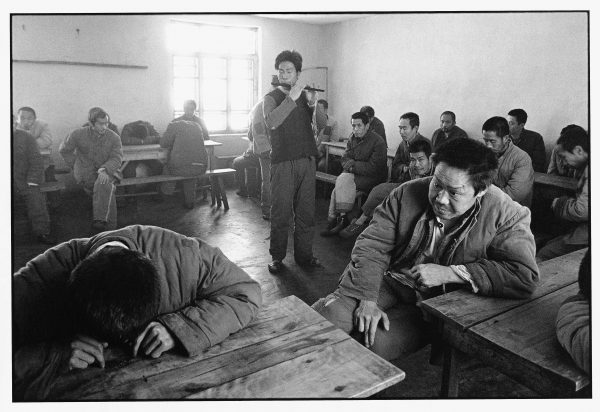1989. 银盐纸基. 27.1×40.6cm. 40.6×50.8cm framed.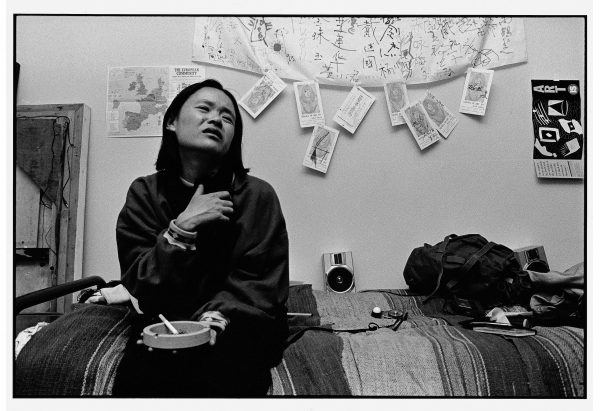1989. 银盐纸基. 27.1×40.6cm. 40.6×50.8cm framed.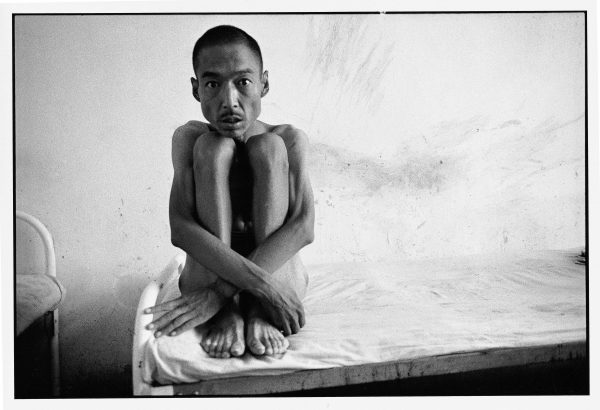1989. 银盐纸基. 27.1×40.6cm. 40.6×50.8cm framed.